# RD Sharma Solutions for Class 11 Maths Chapter 16 Permutations

## RD Sharma Solutions Class 11 Maths Chapter 16 – Download Free PDF Updated for 2021-22

RD Sharma Solutions for Class 11 Maths Chapter 16 – Permutations are provided here for students to score good marks in the board exams. “The number of possible combinations of ‘r’ objects from a set on ‘n’ objects.” So, permutations are used for lists (in which order matters) and Combination for groups (order doesn’t matter). In this chapter, we shall introduce the term and notation of a factorial. RD Sharma Solutions module employs numerous shortcut tips and illustrations to explain all the exercise problems in the most understandable language.

The subject experts at BYJU’S have simplified the difficult problems into simpler steps, which can be easily solved by students with precision. RD Sharma Class 11 Maths Solutions will help students in obtaining knowledge and strong command over the subject. Hence, students are advised to practice the solutions by downloading the pdf available from the links given below.

Chapter 16 – Permutations contains five exercises and the RD Sharma Solutions present in this page provide solutions to the questions present in each exercise. Now, let us have a look at the concepts discussed in this chapter.

• The factorial.
• Fundamental principles of counting.
• Permutations.
• Permutations under certain conditions.
• Permutations of objects not all distinct.

## Download the pdf of RD Sharma Solutions for Class 11 Maths Chapter 16 – Permutations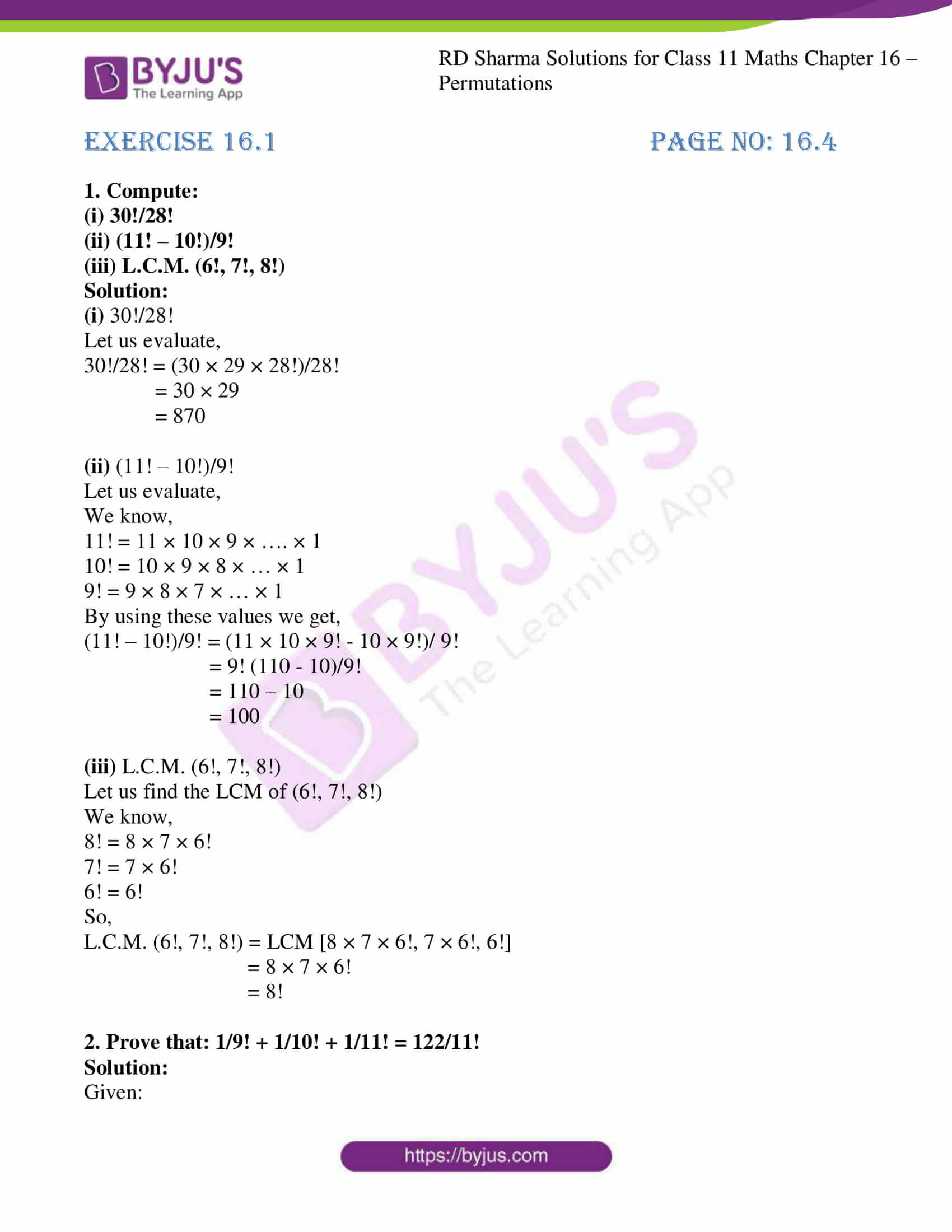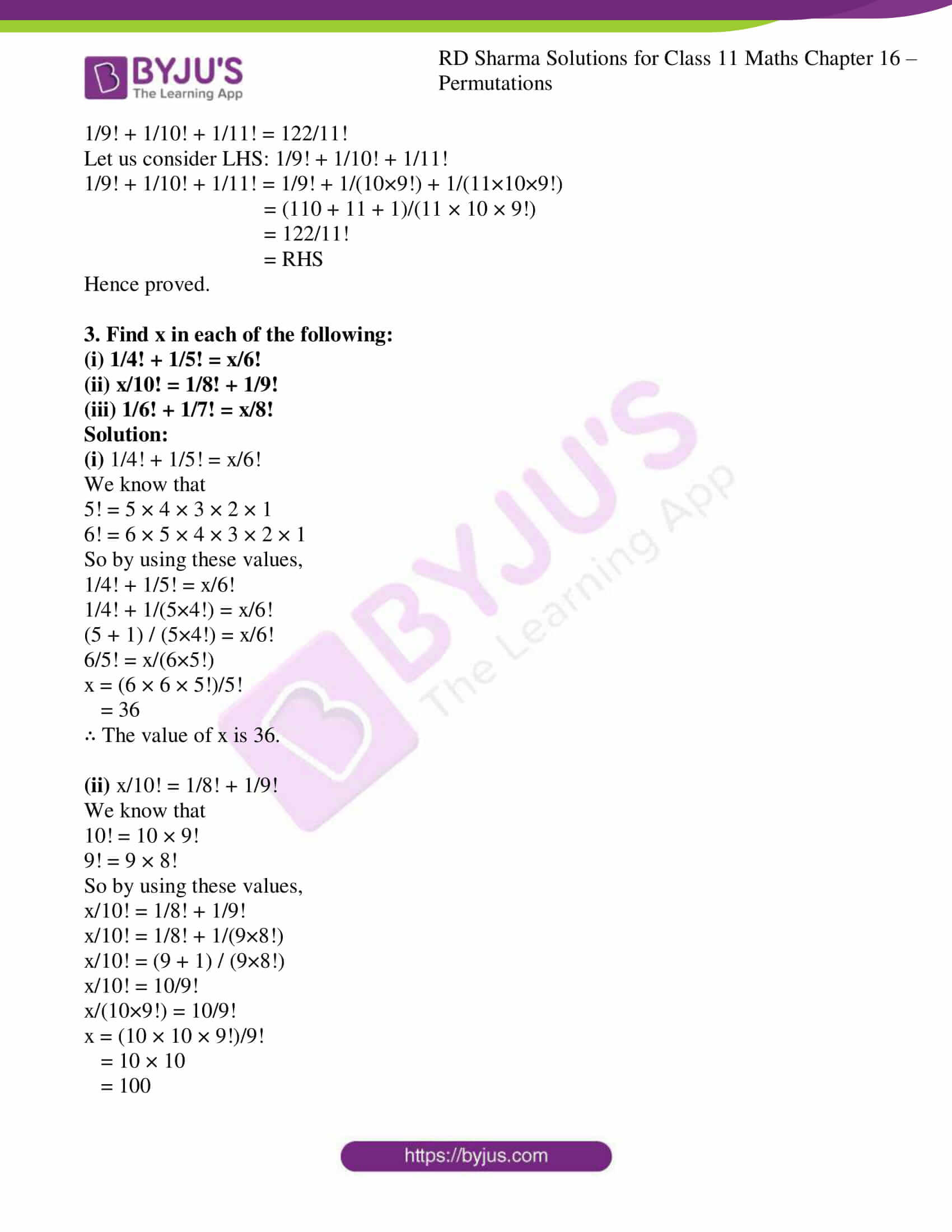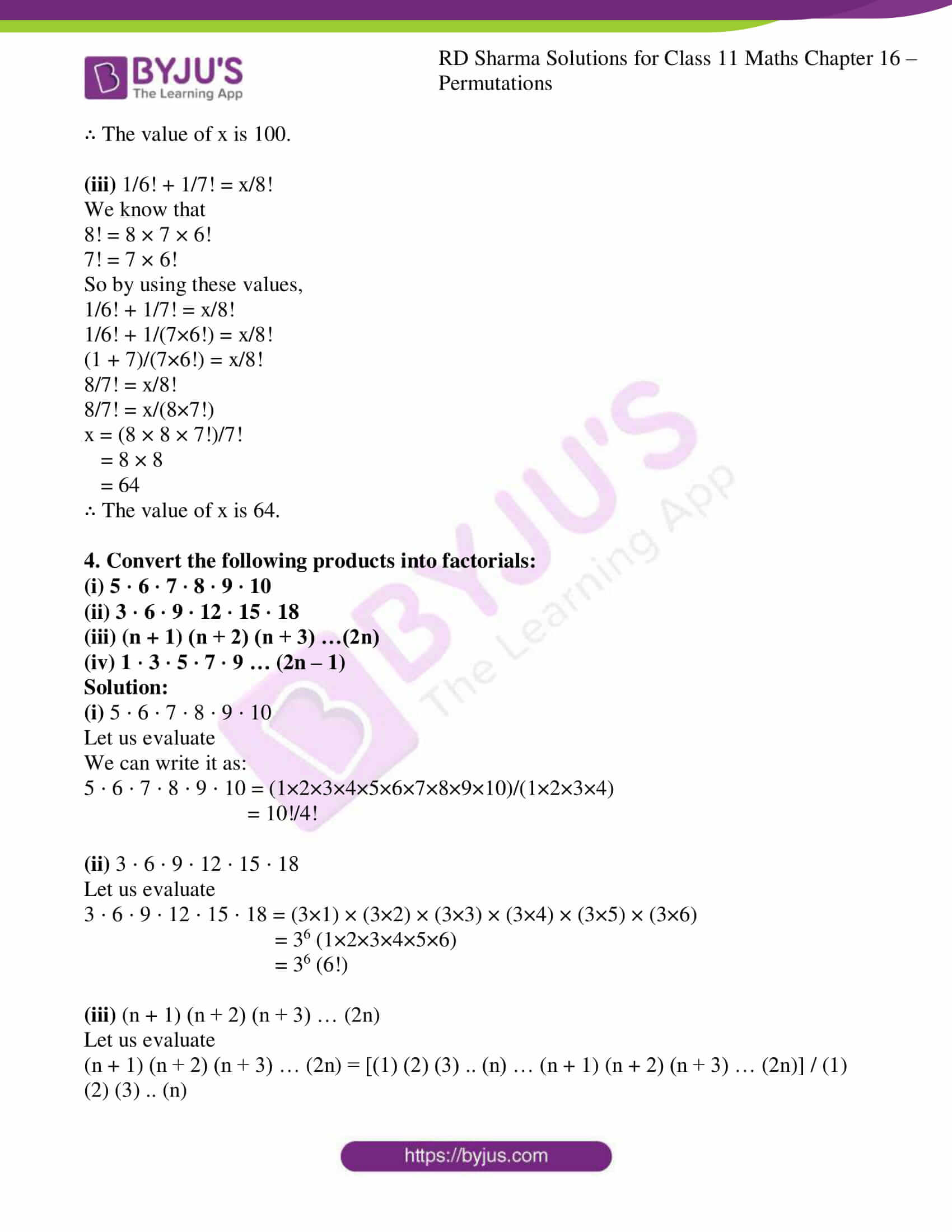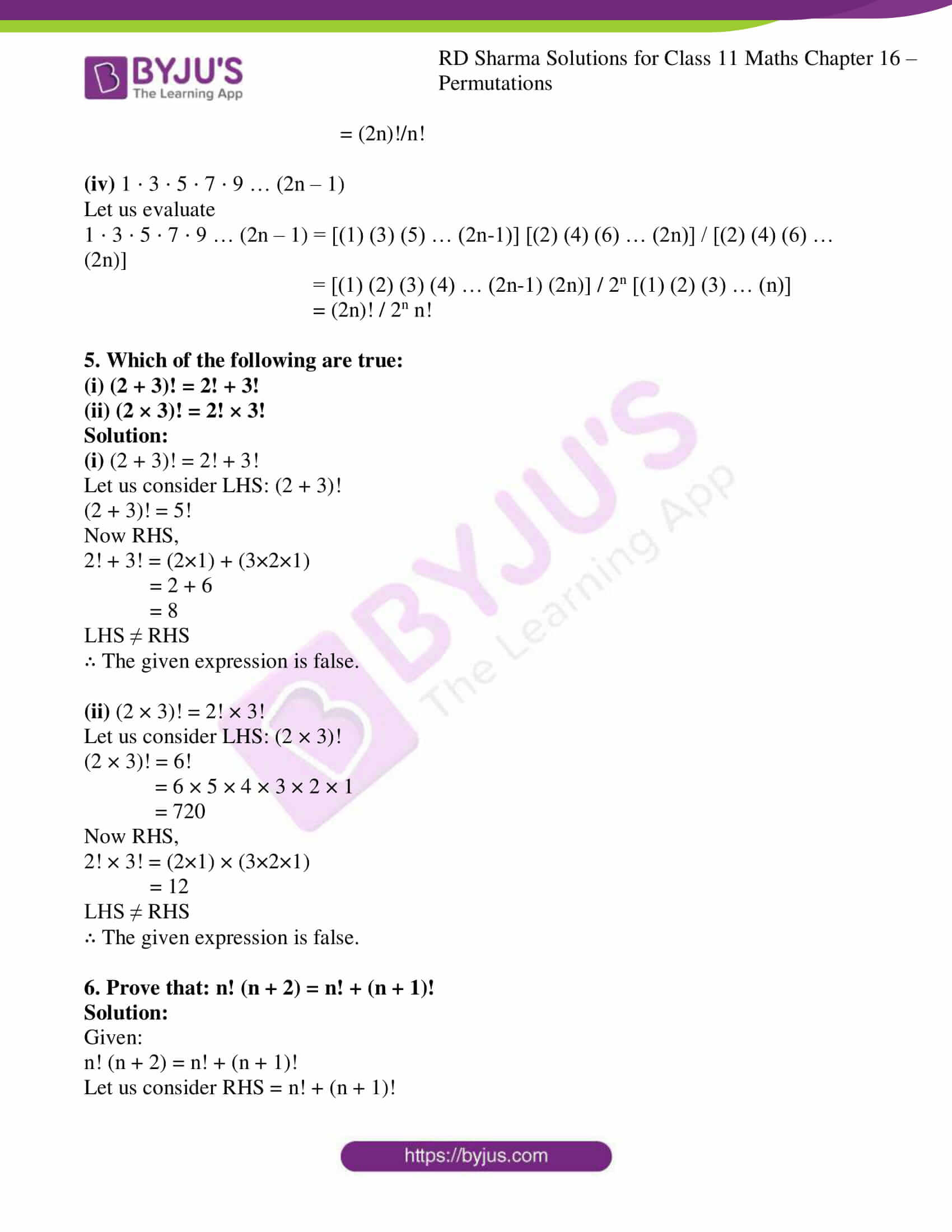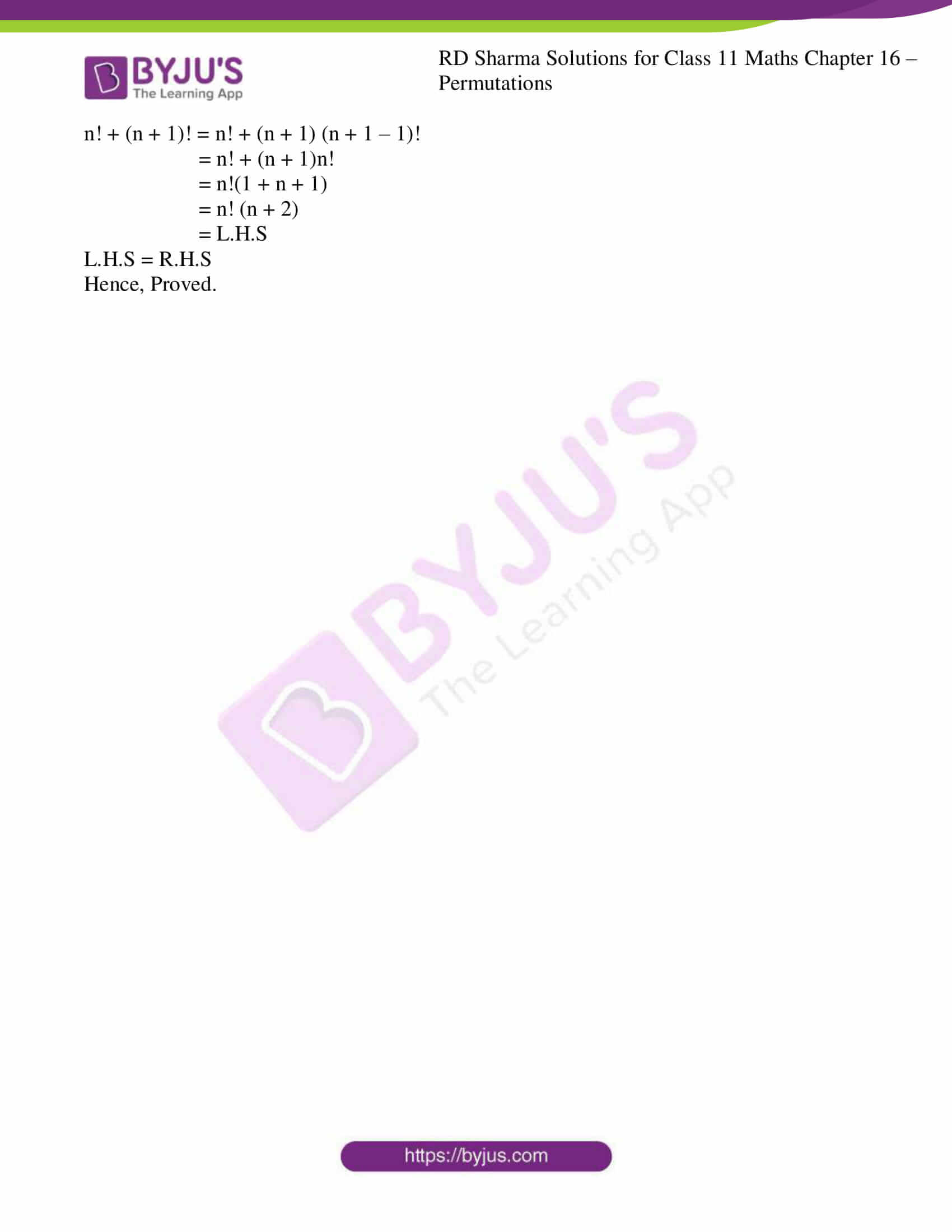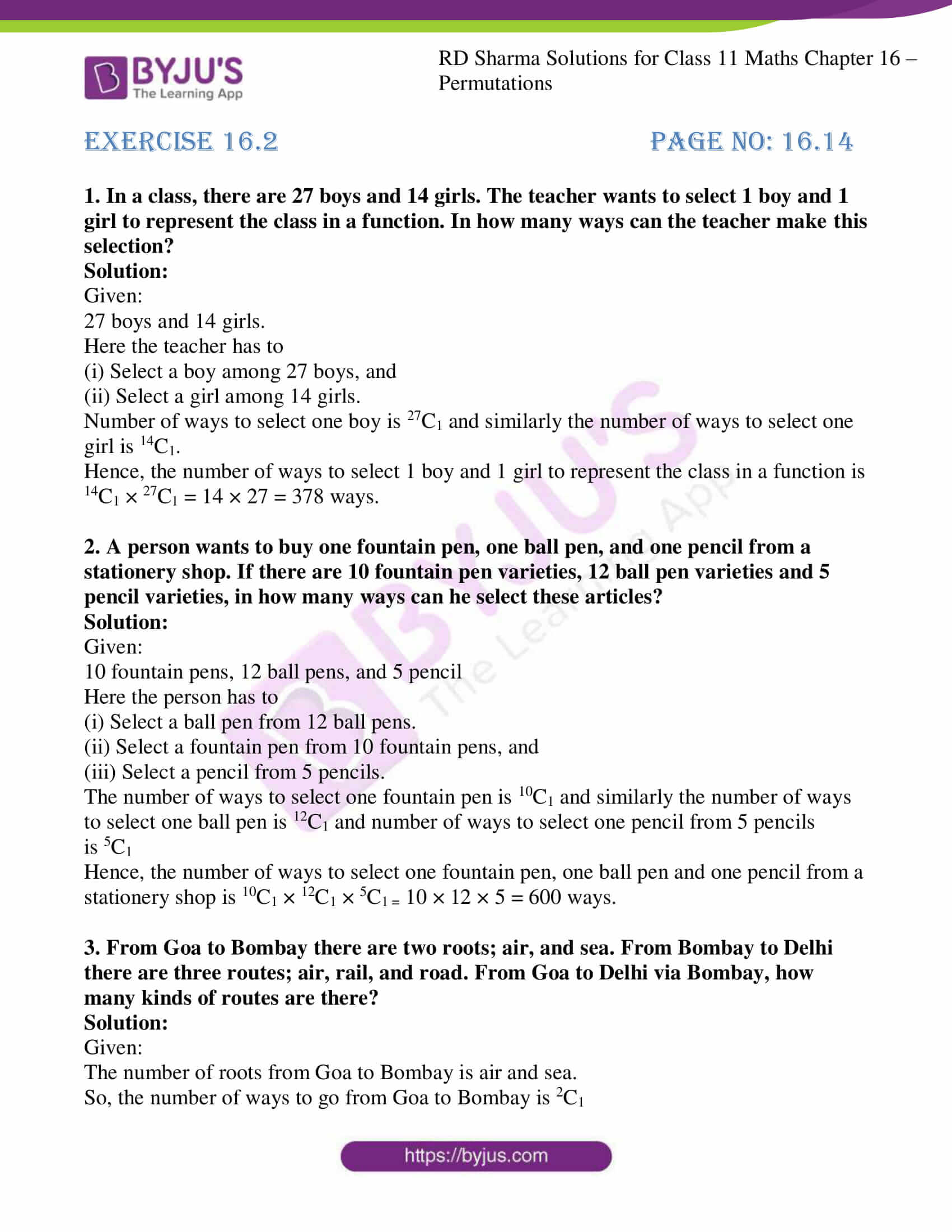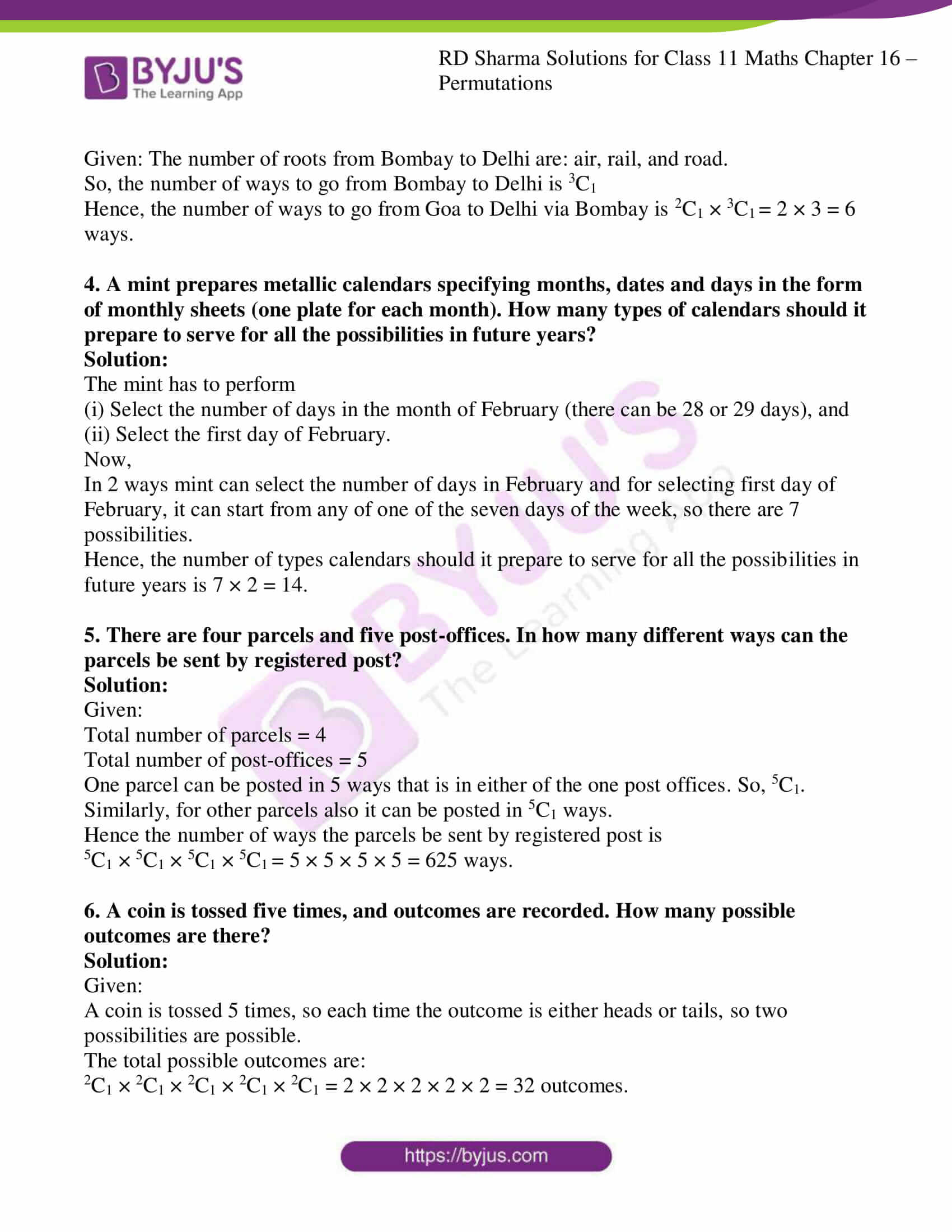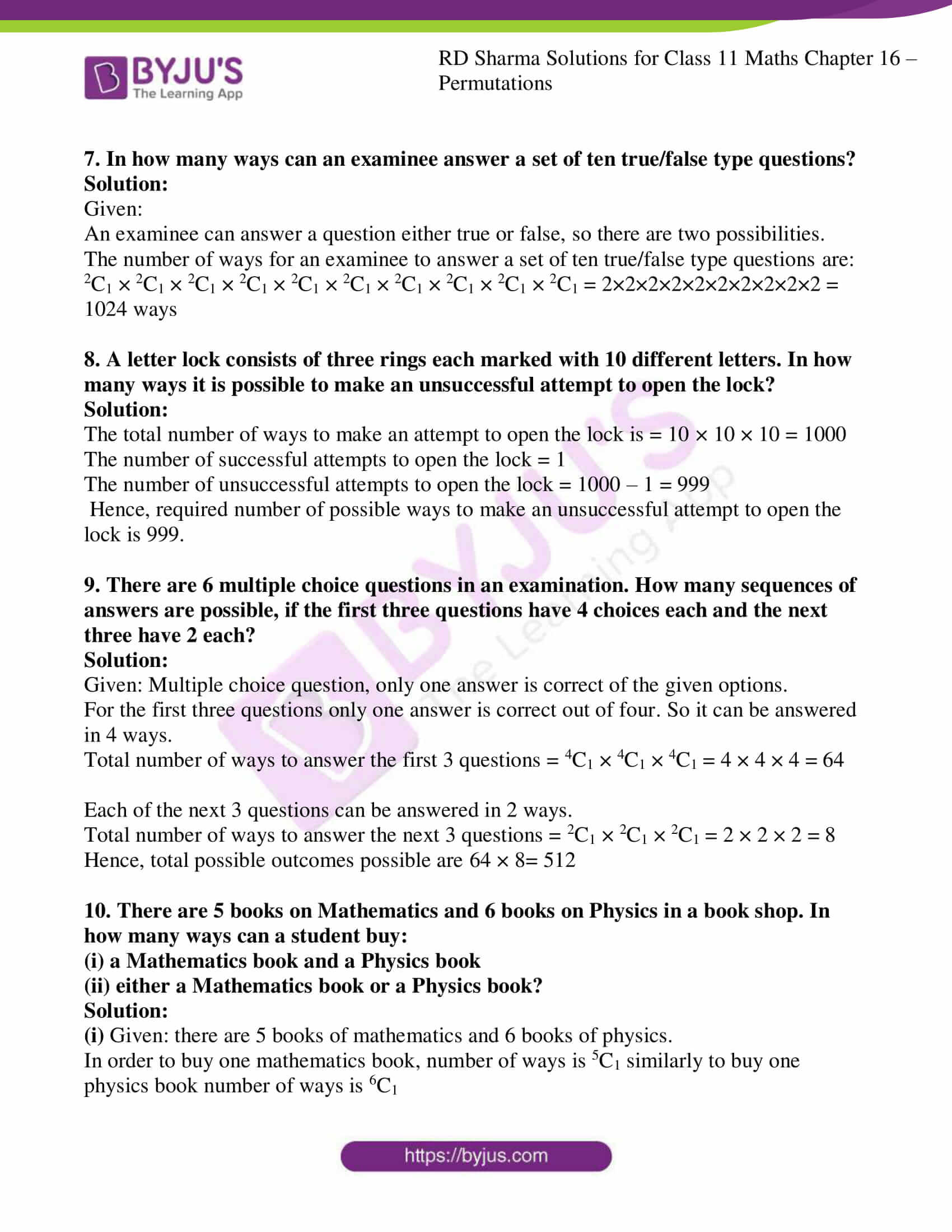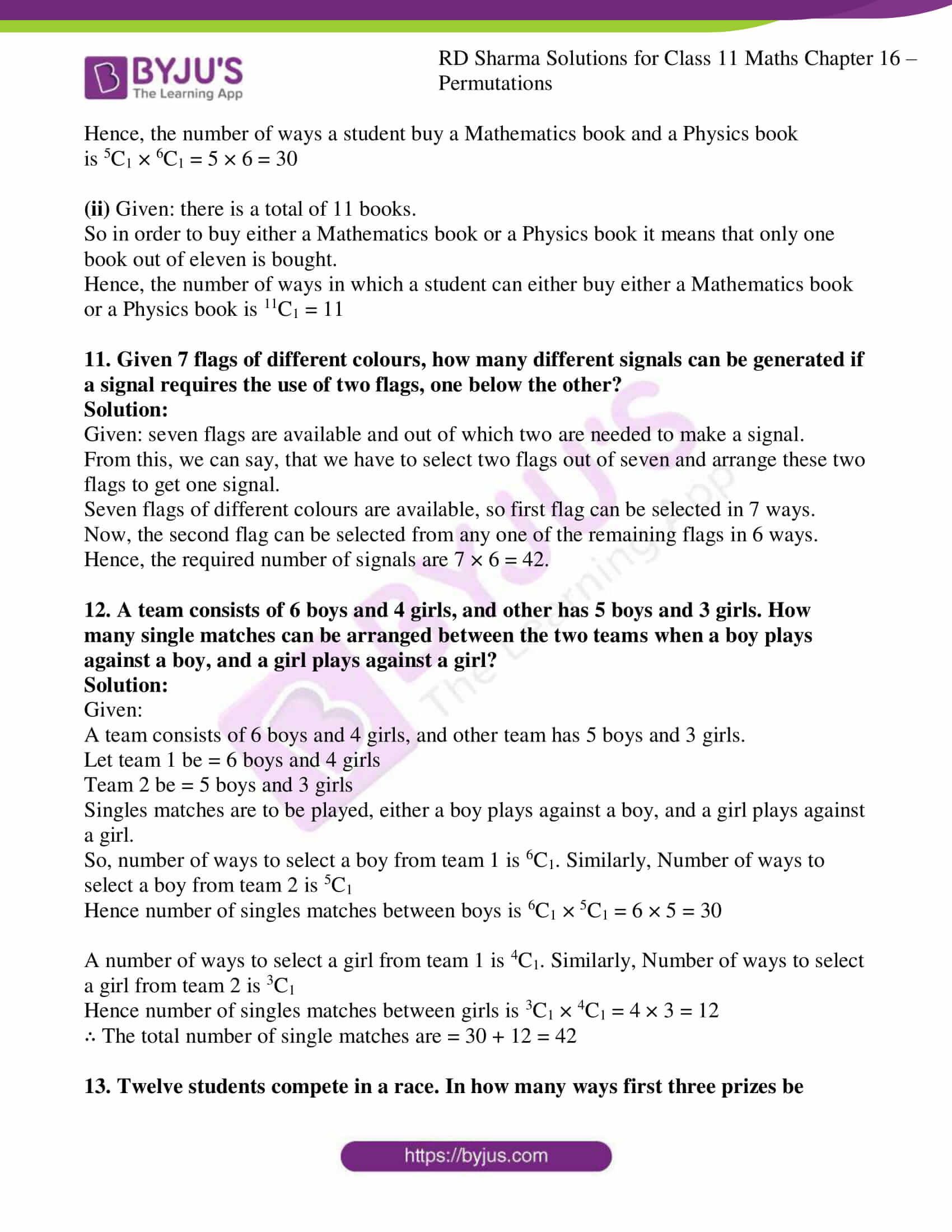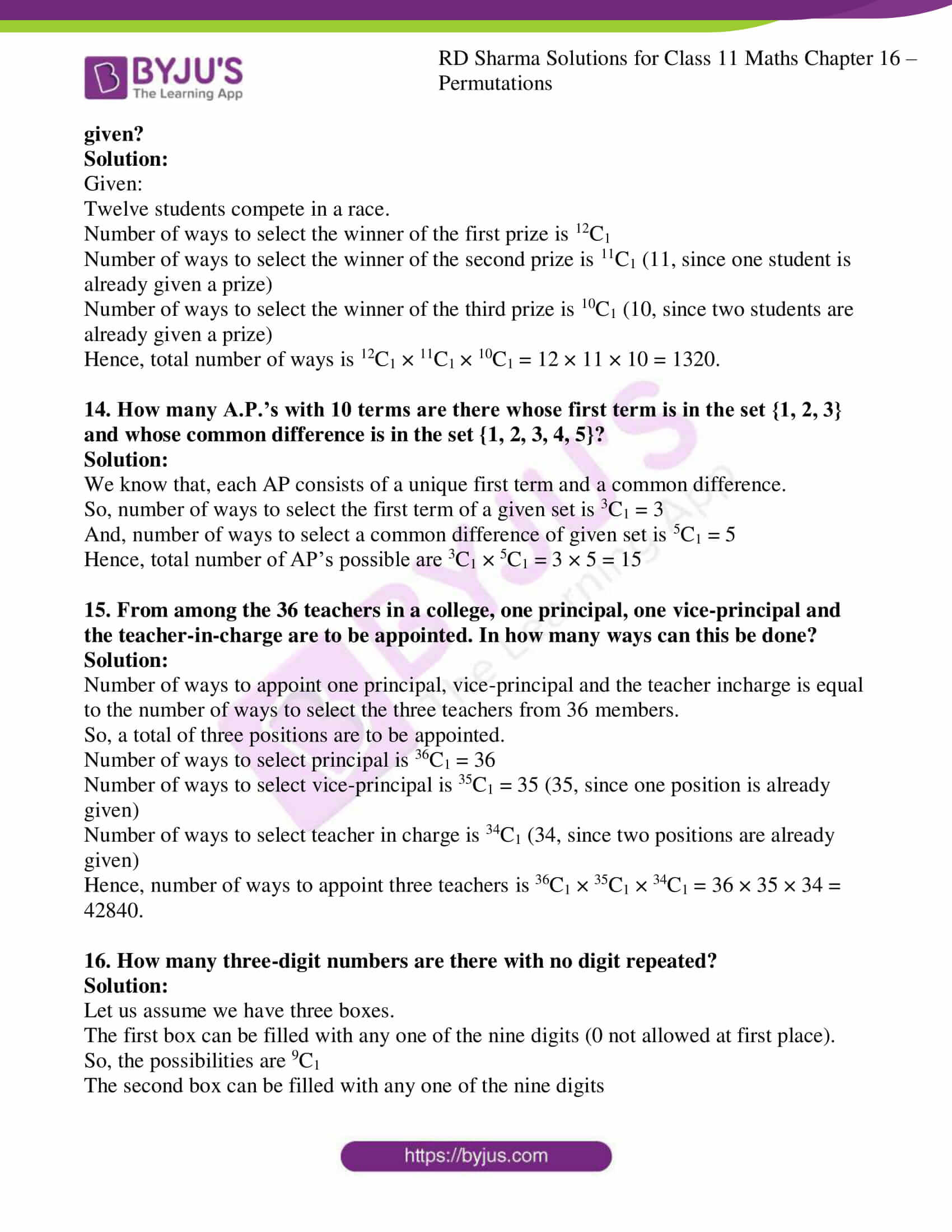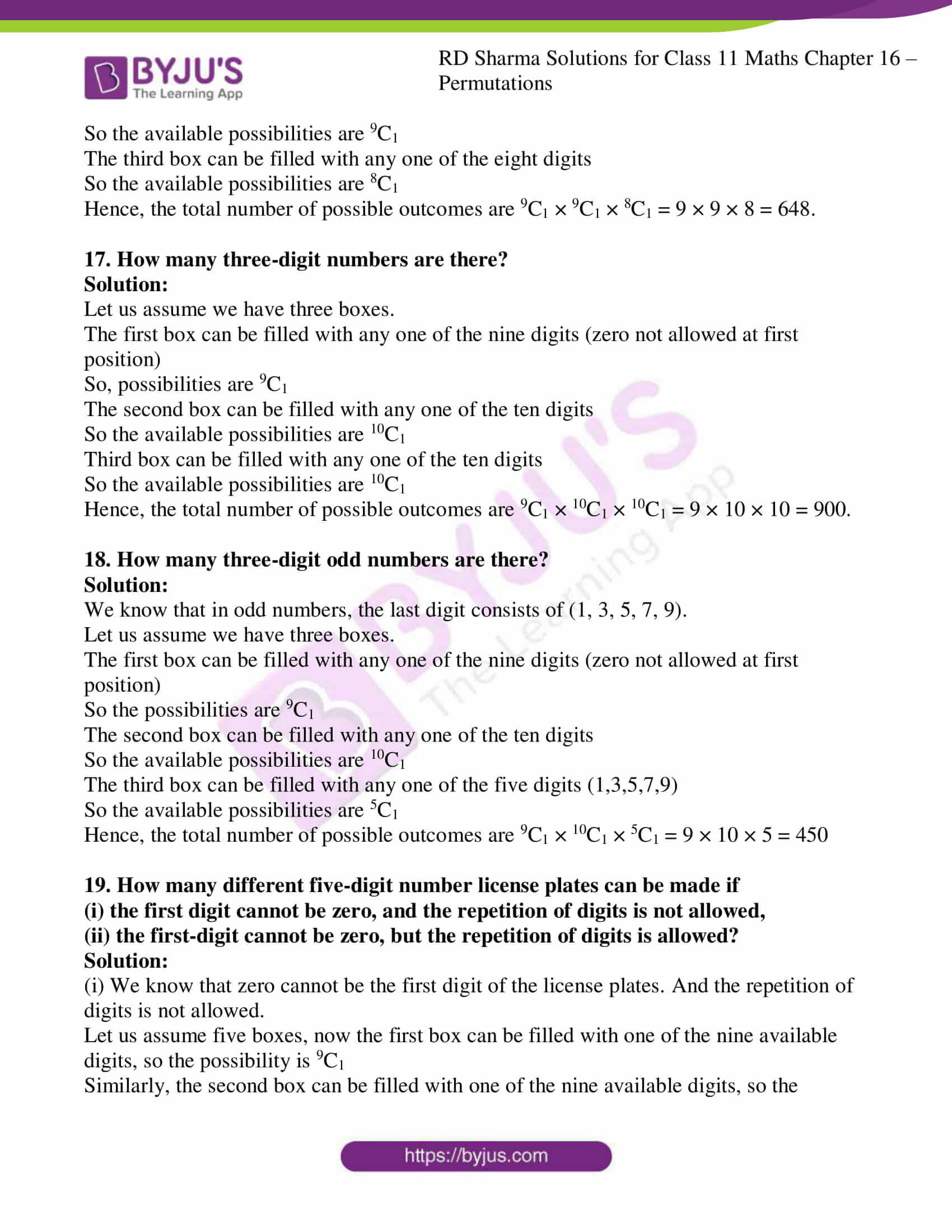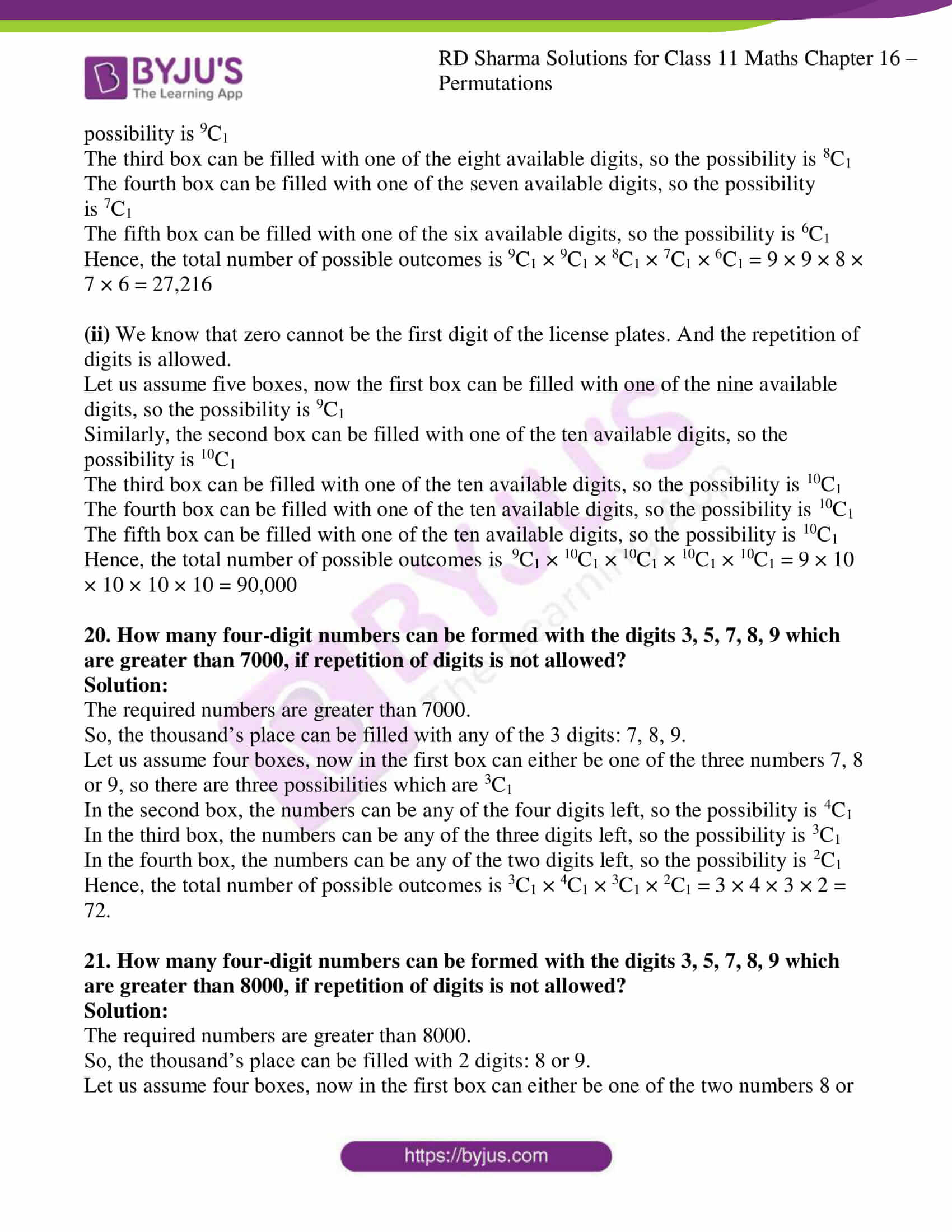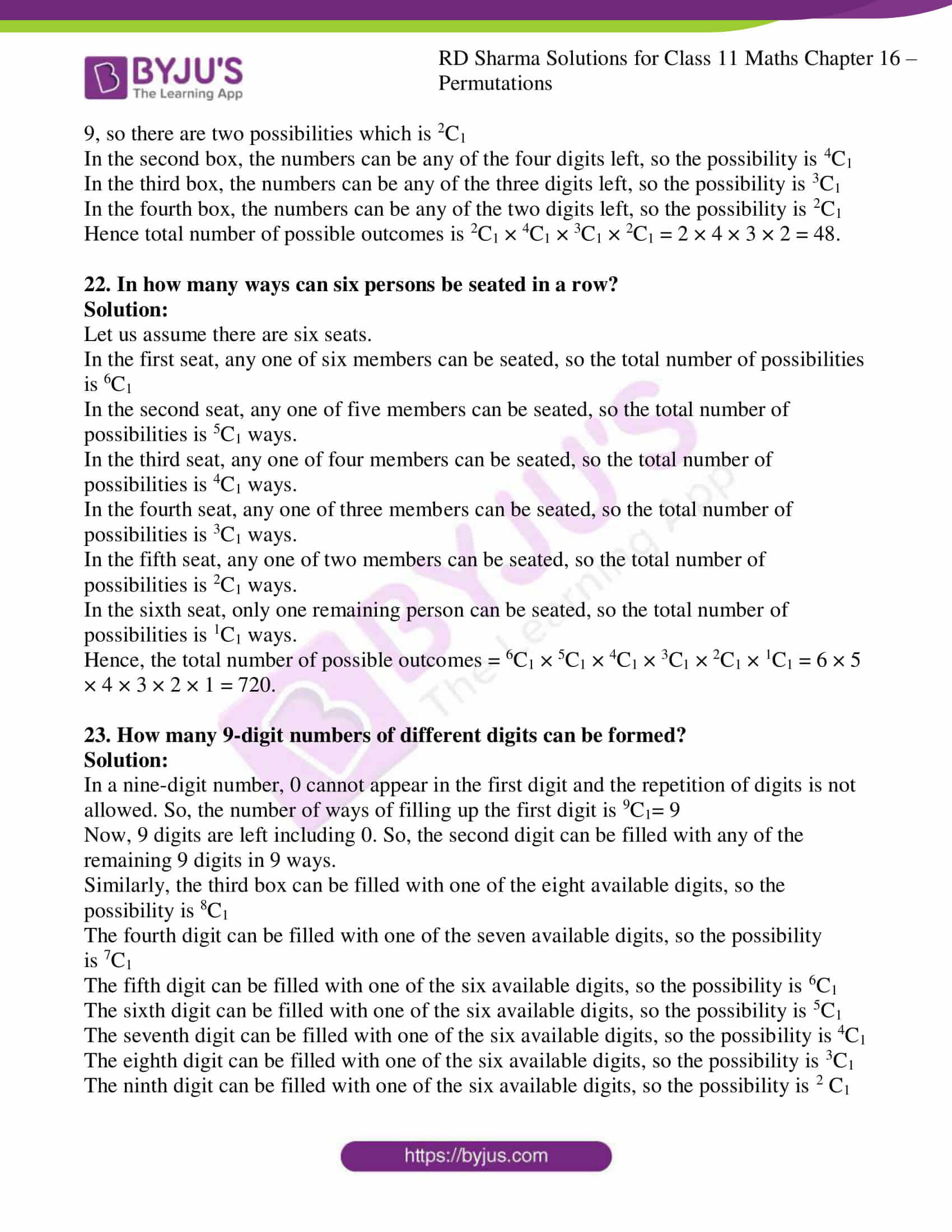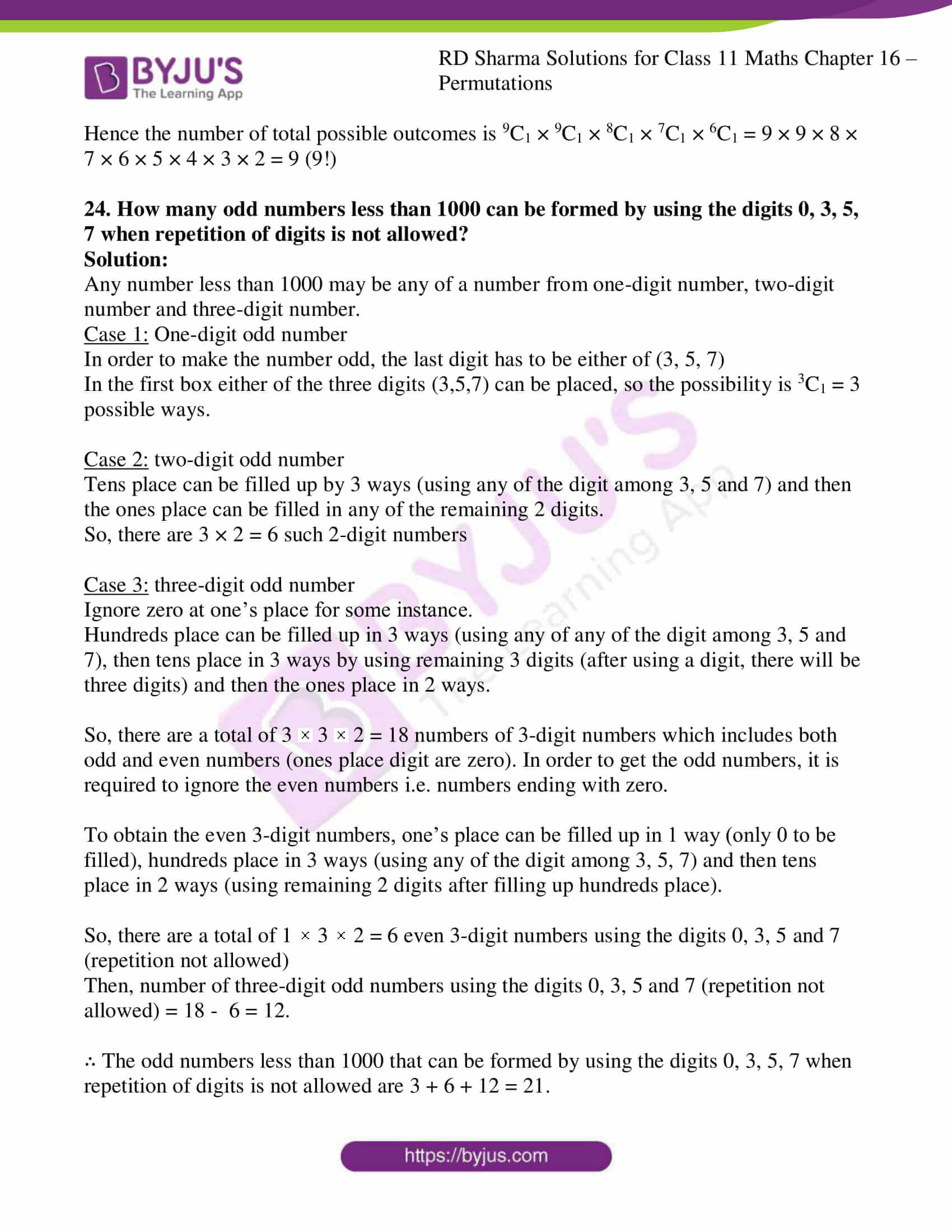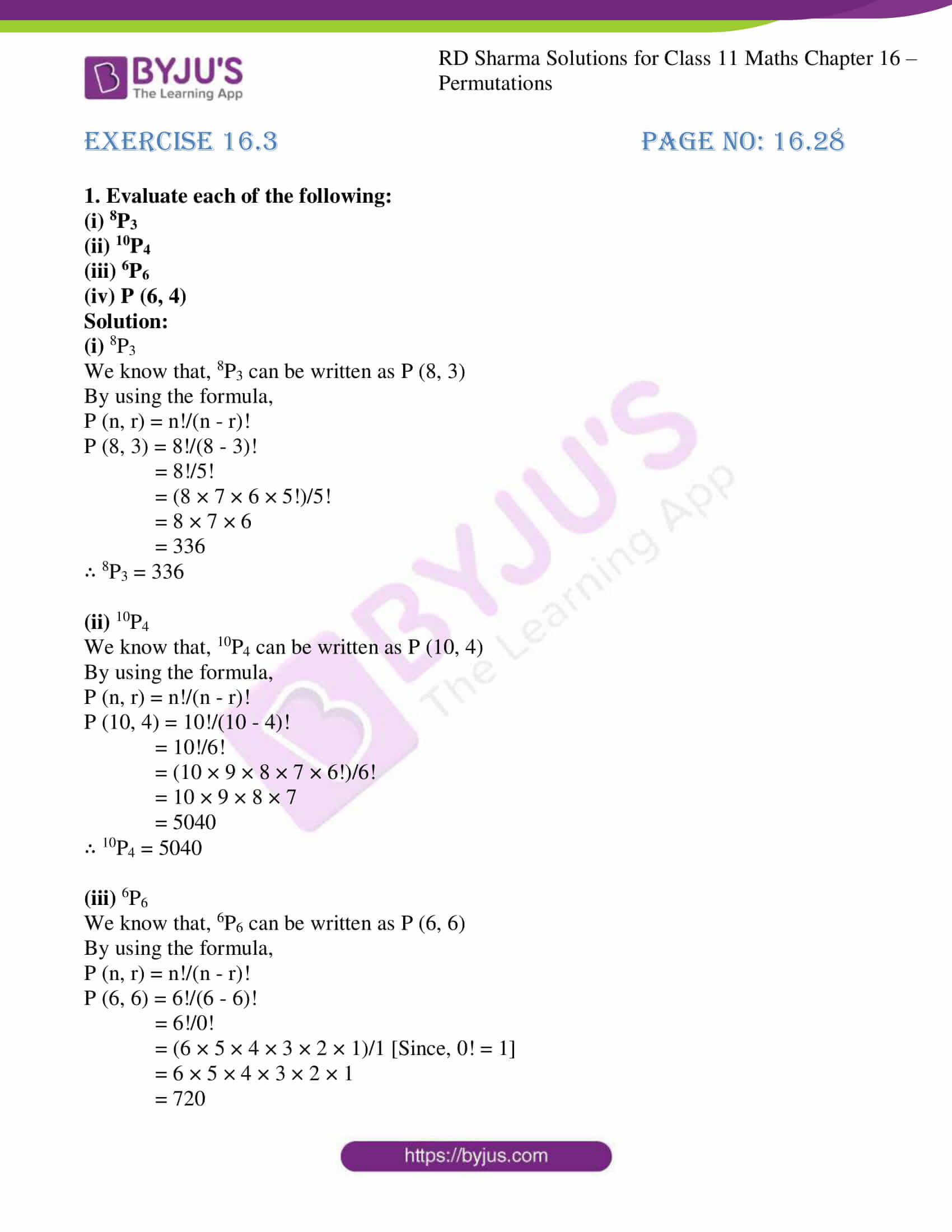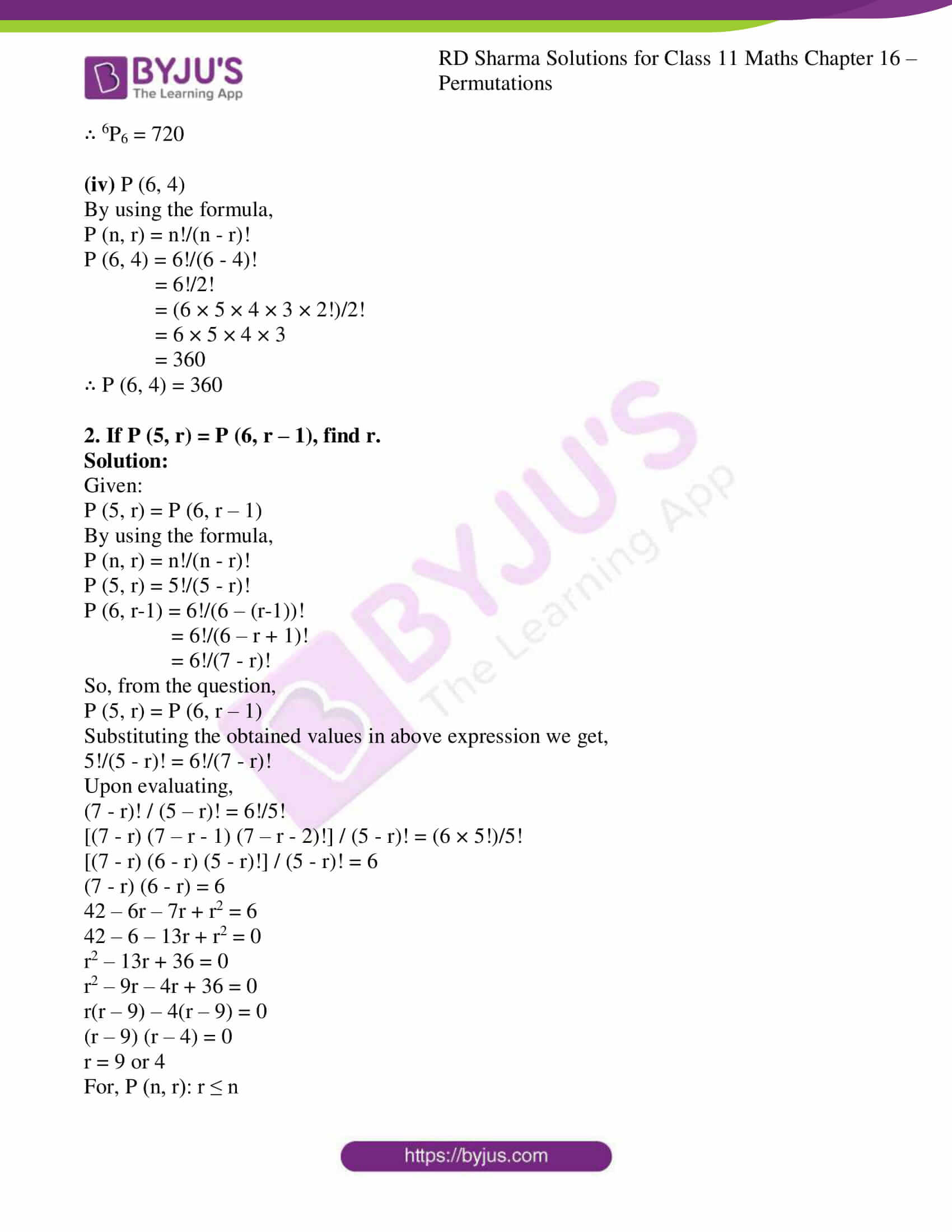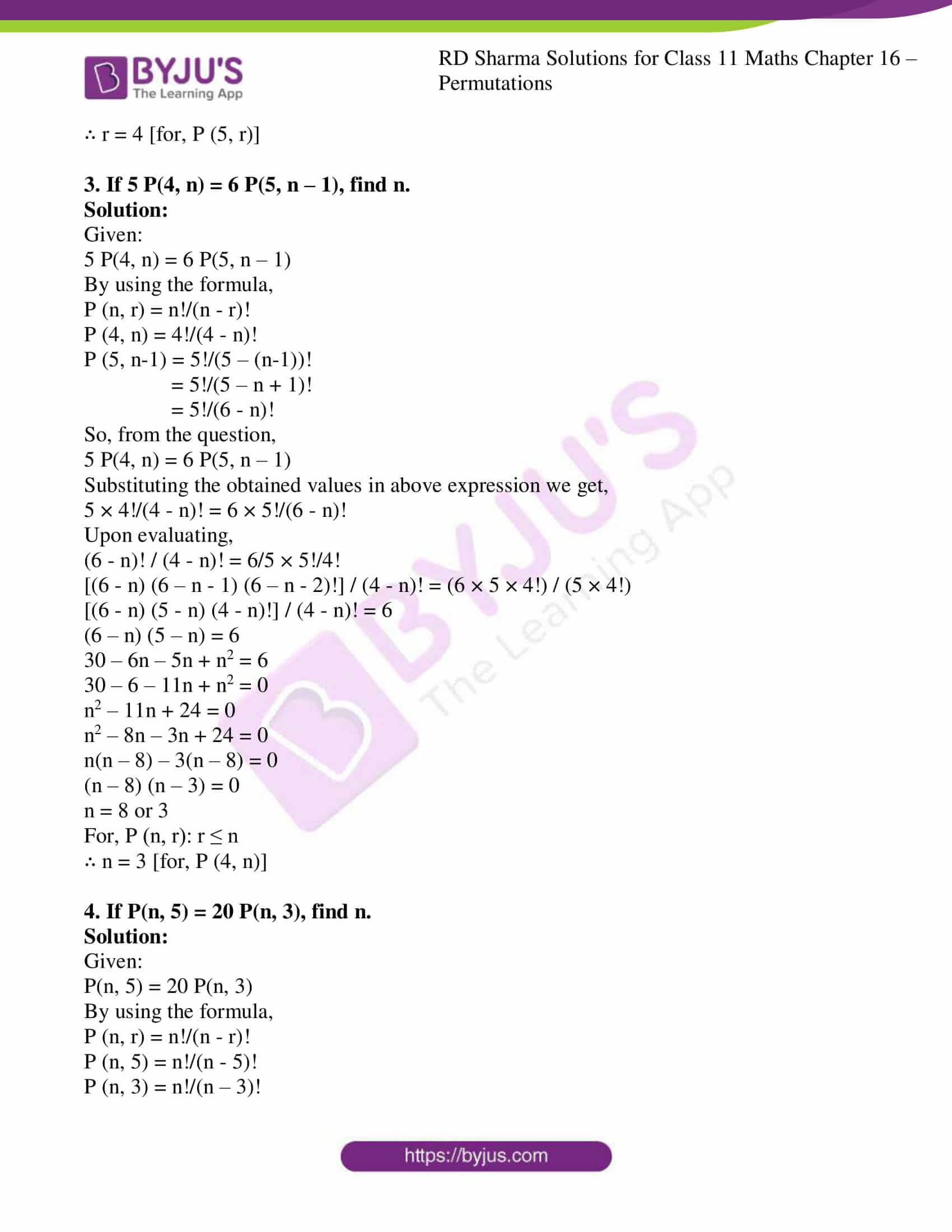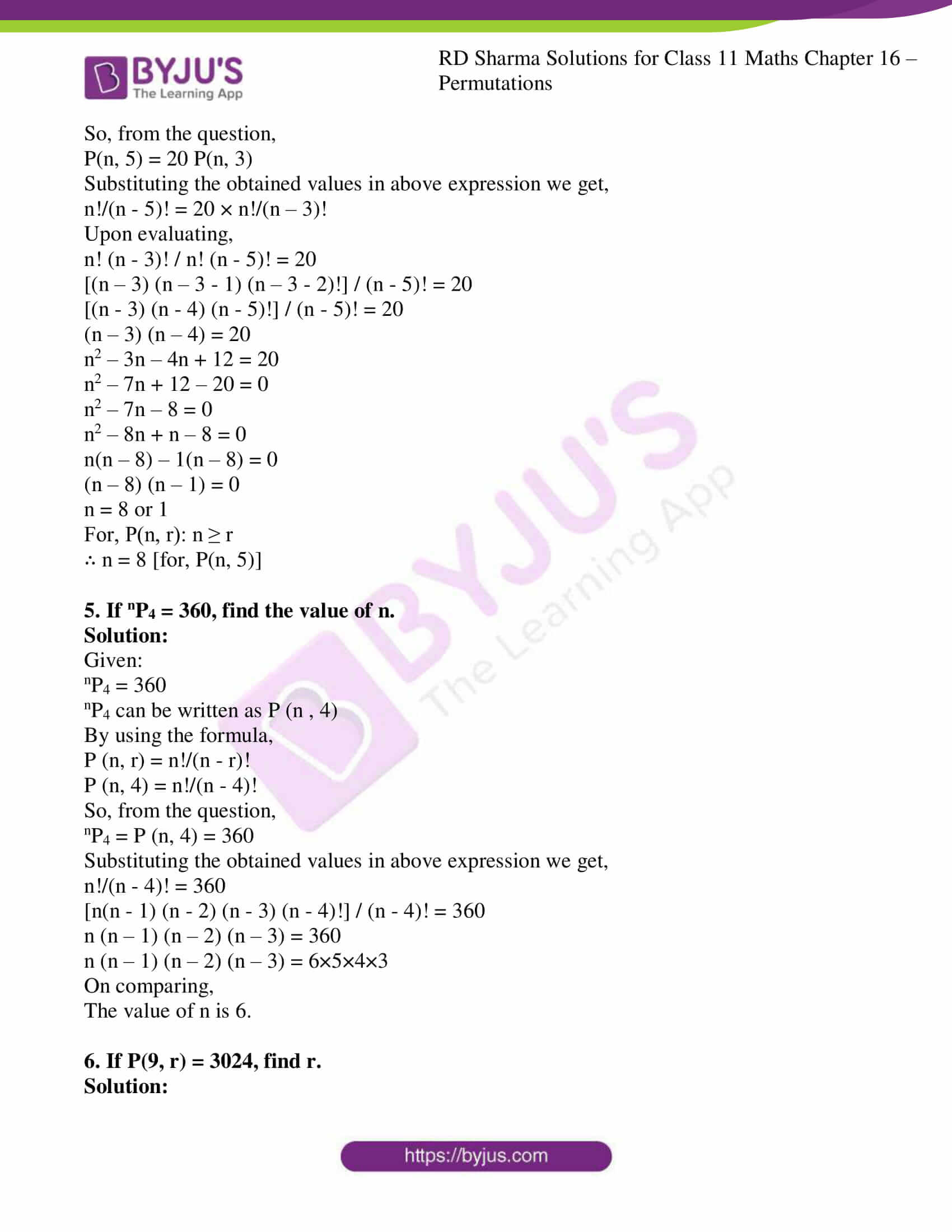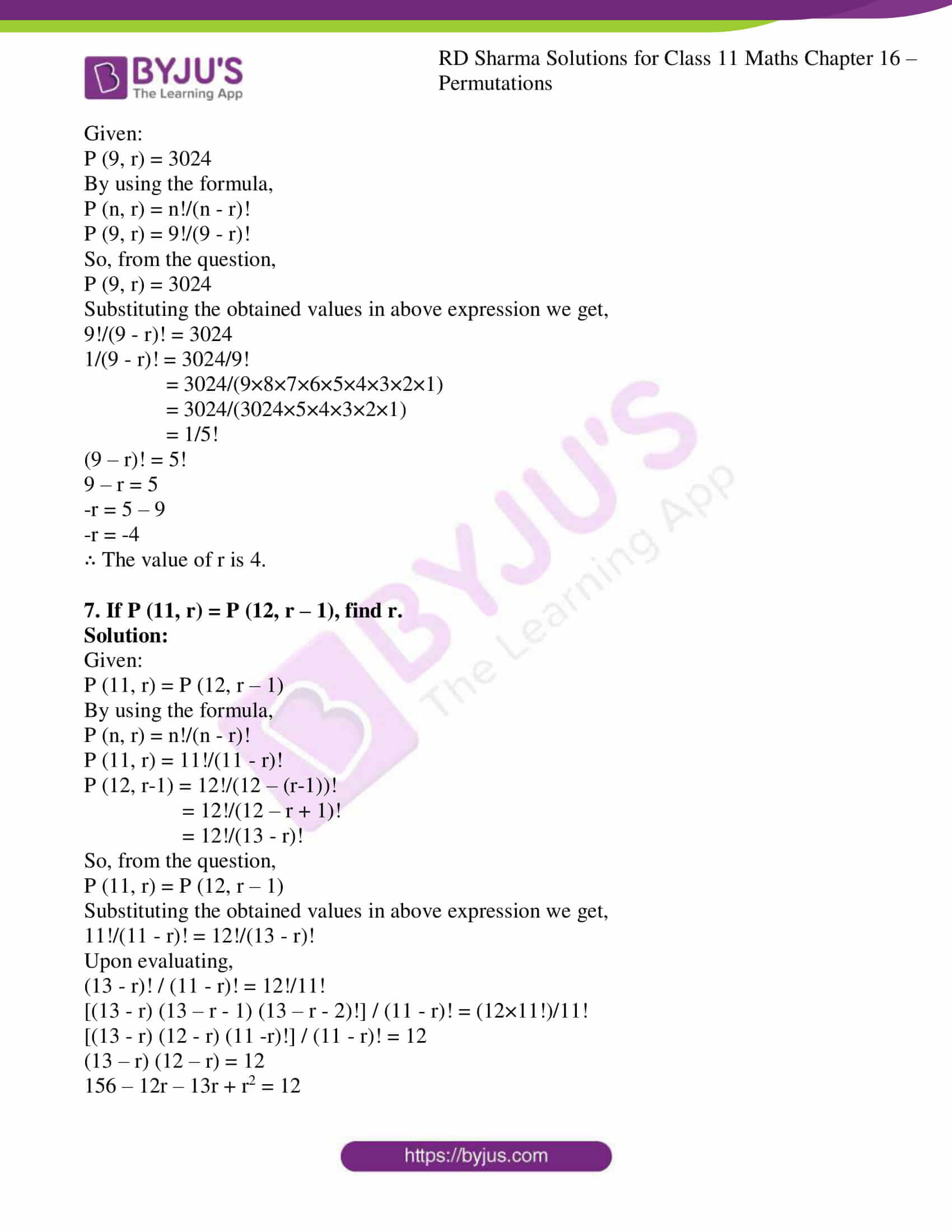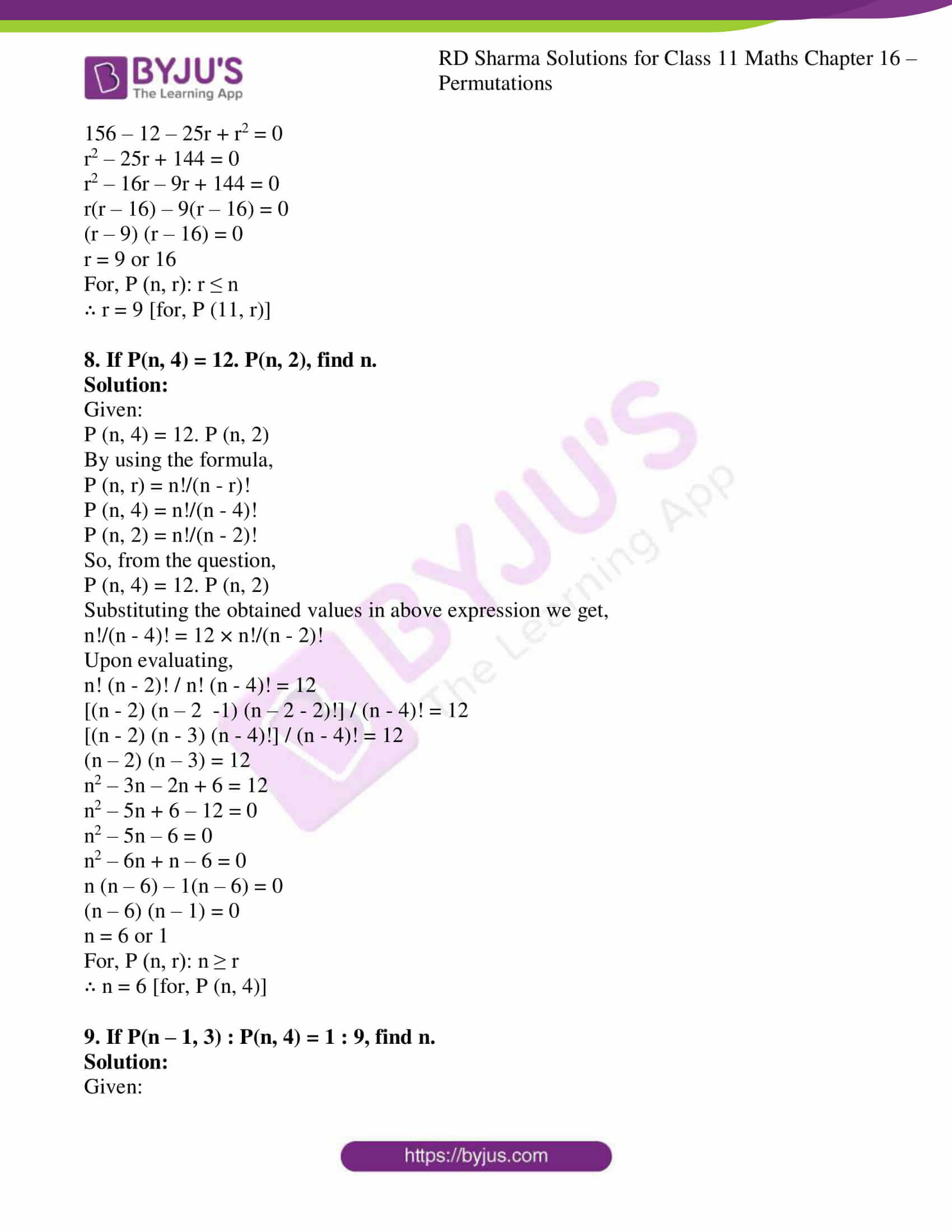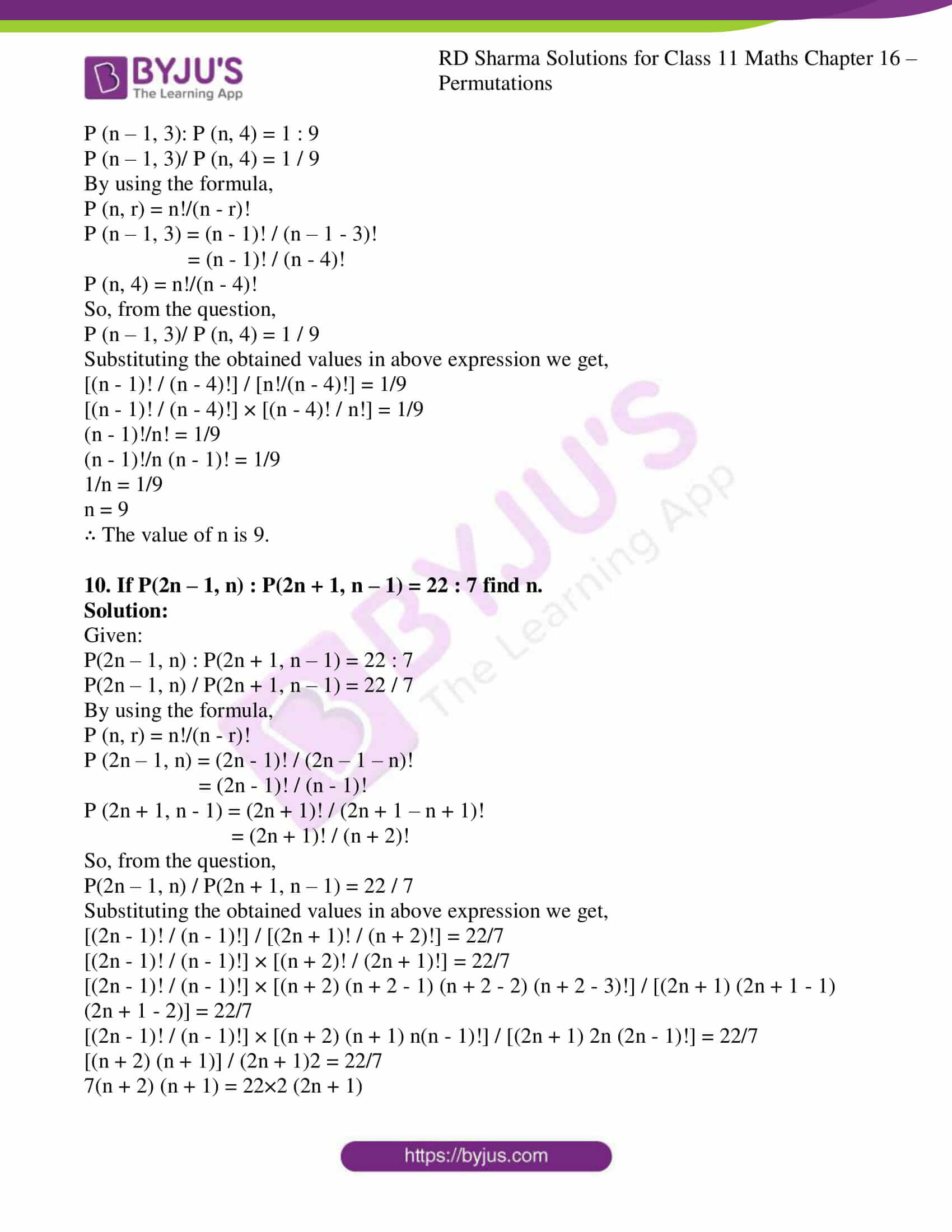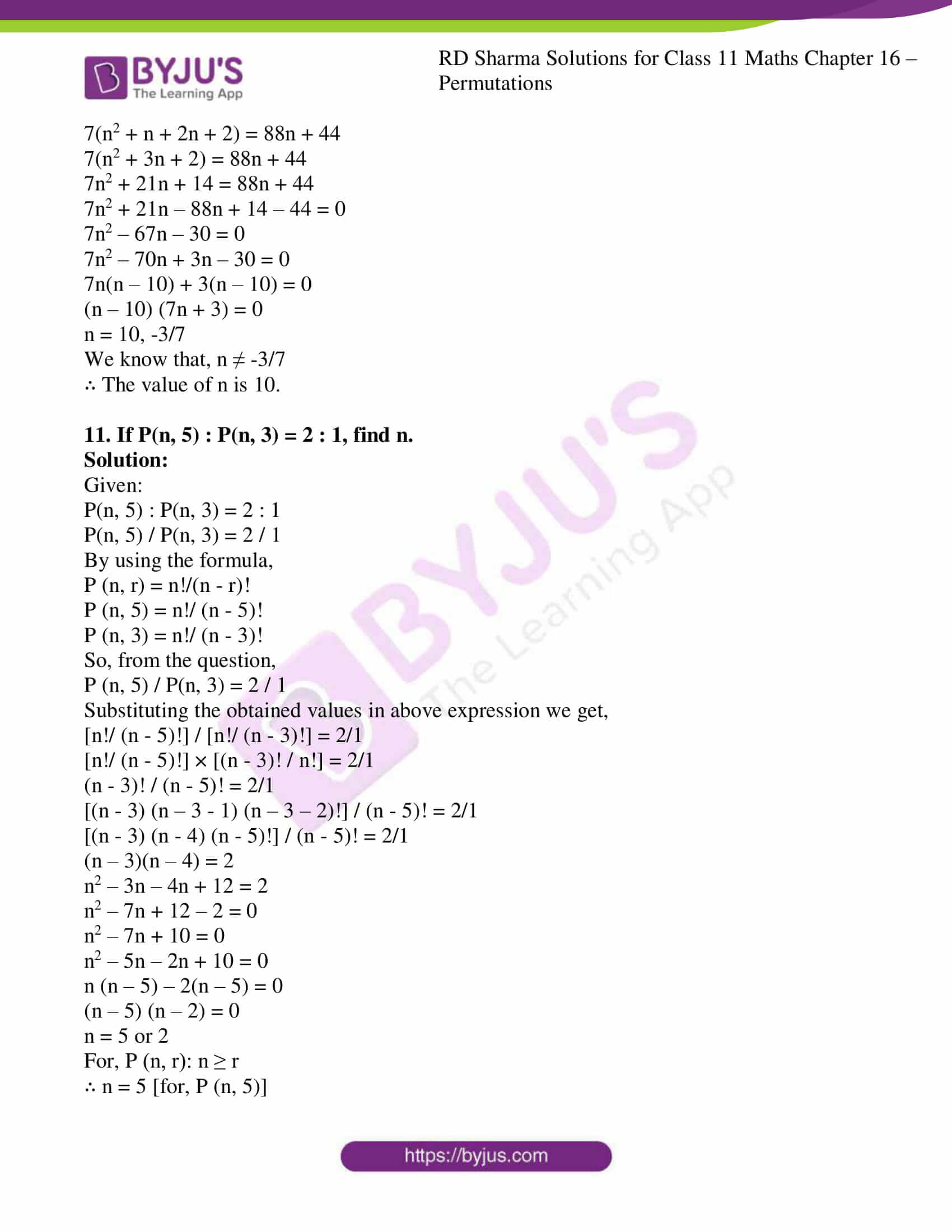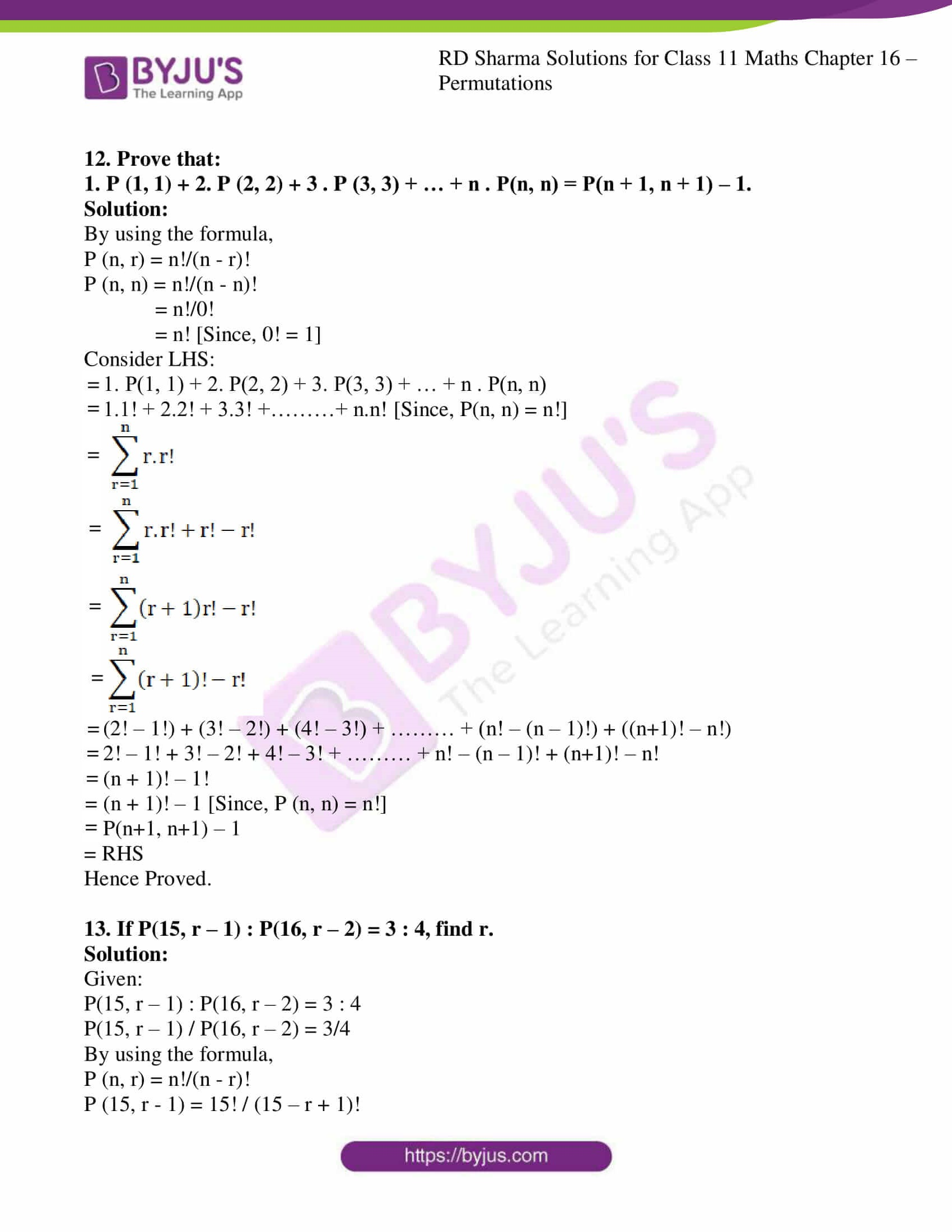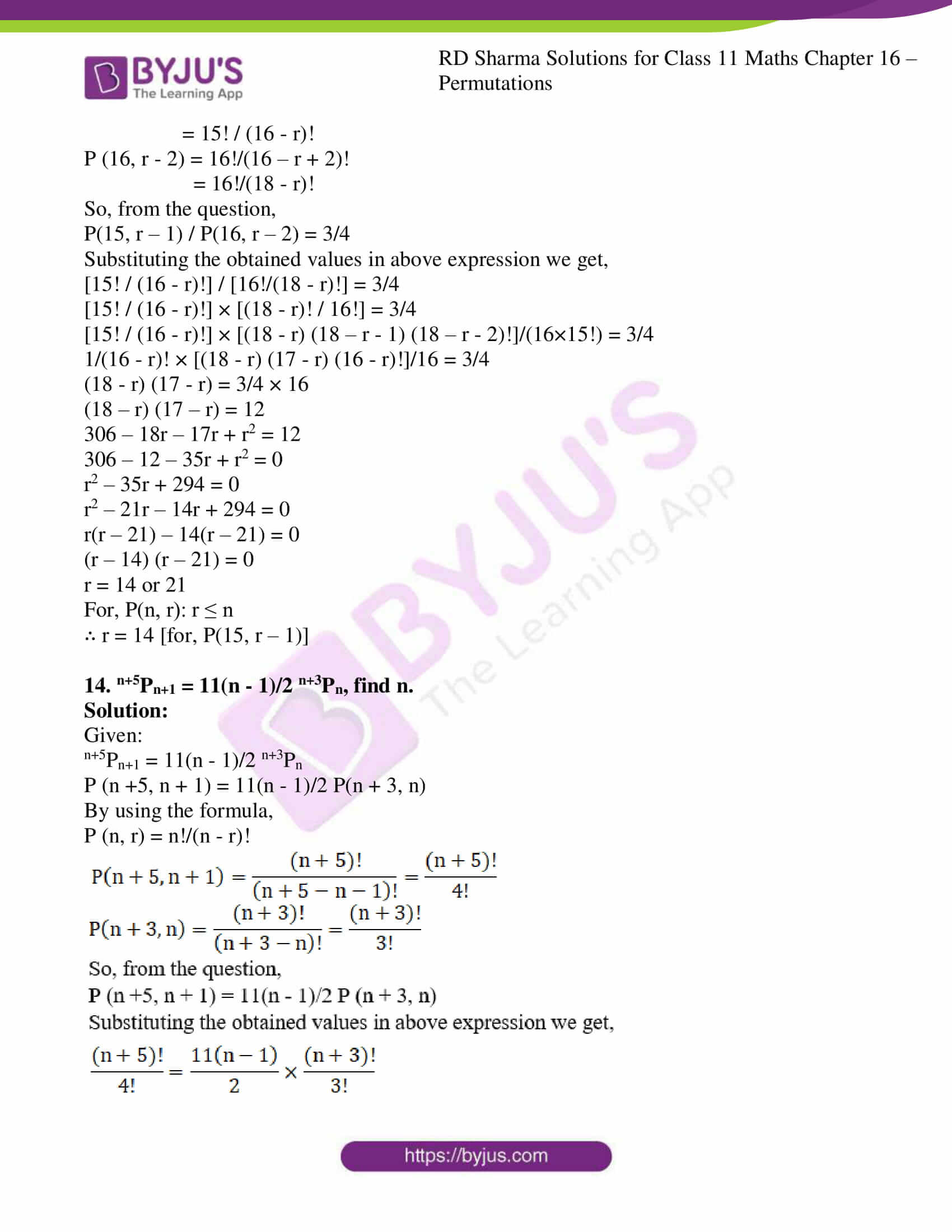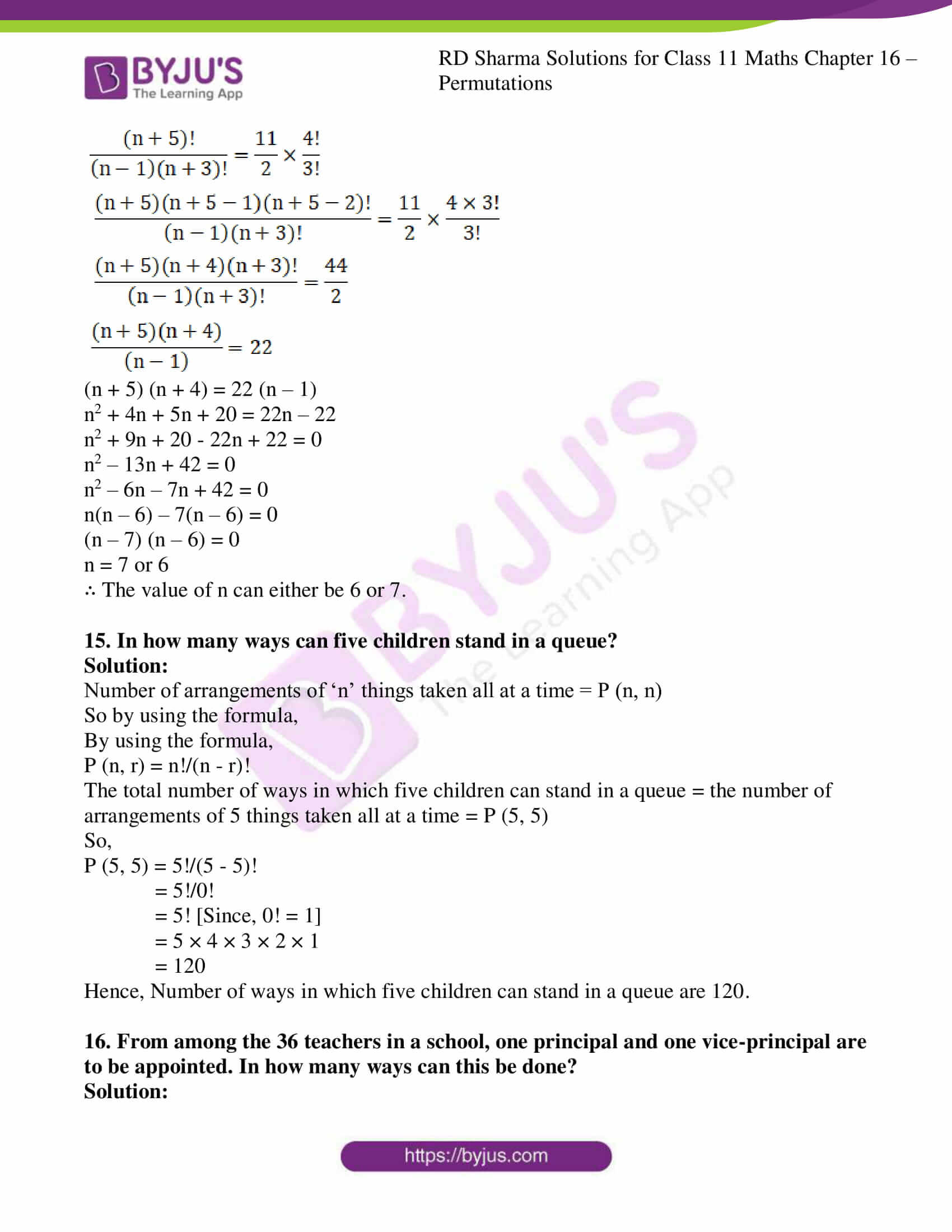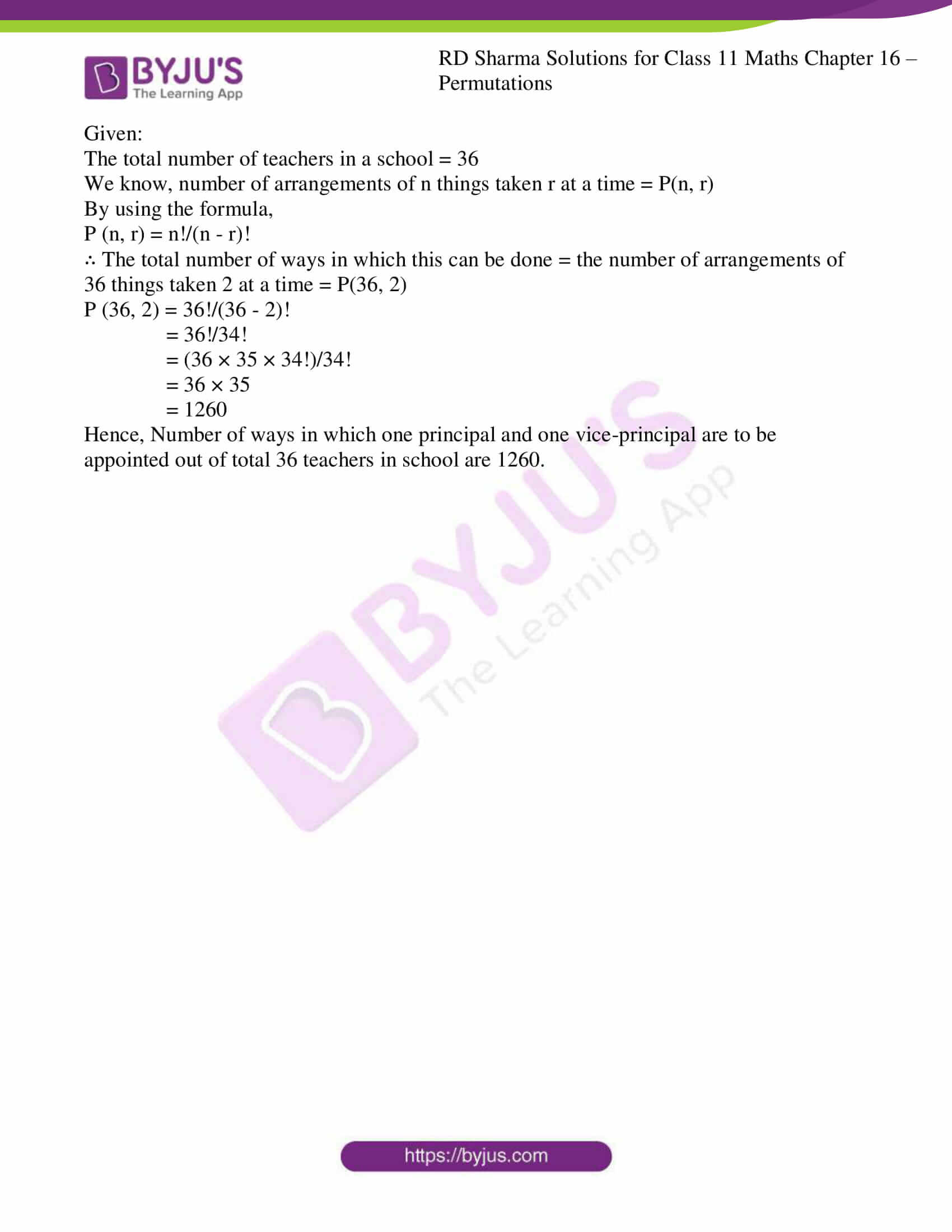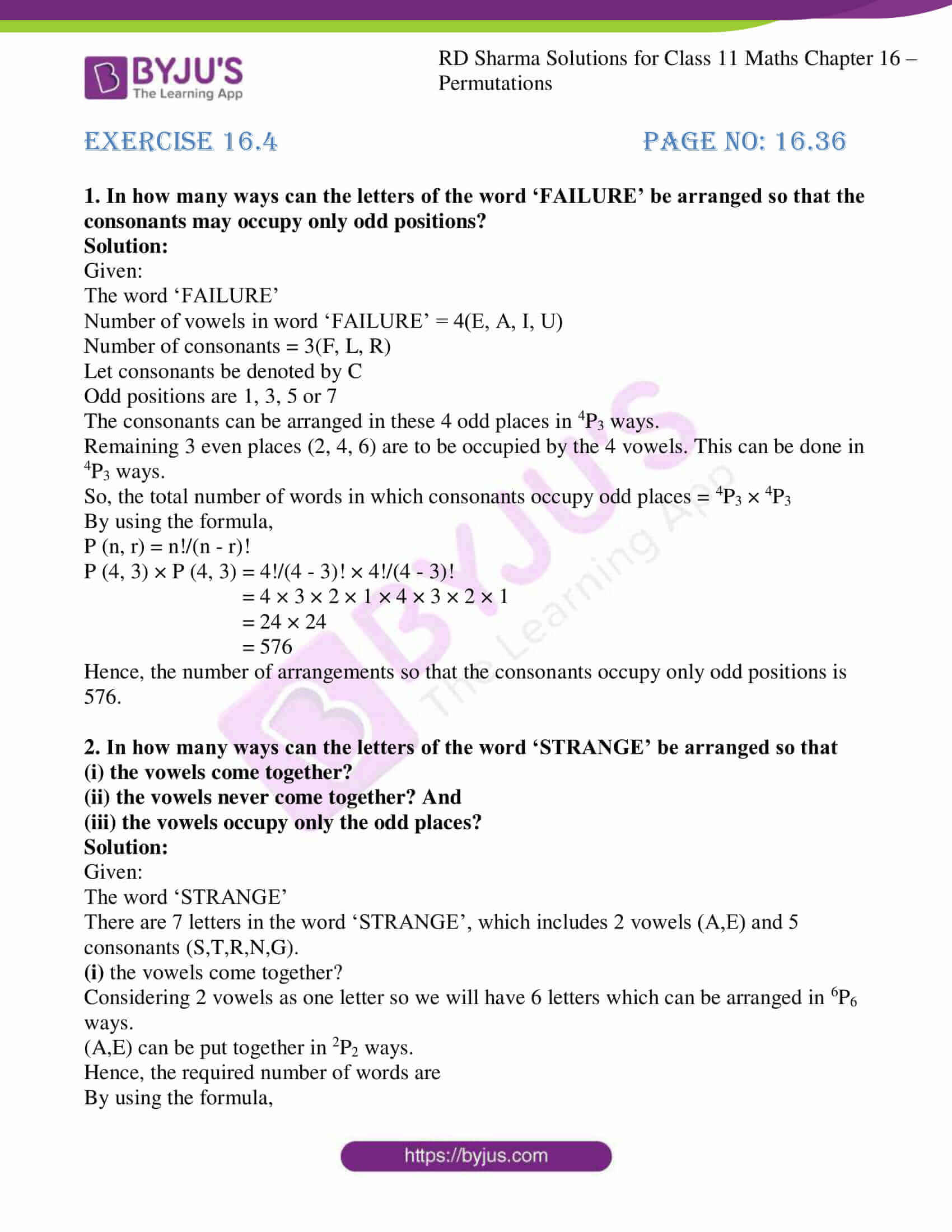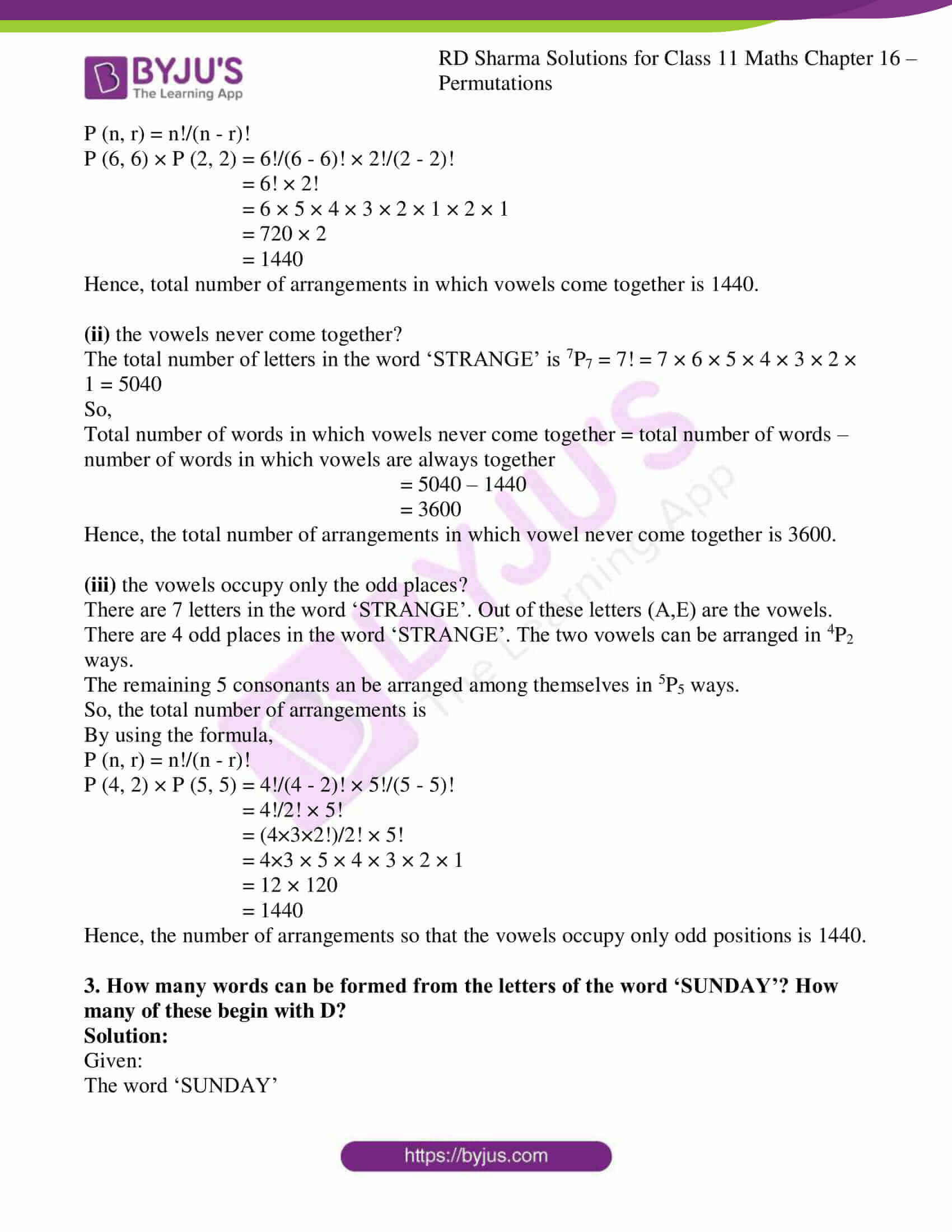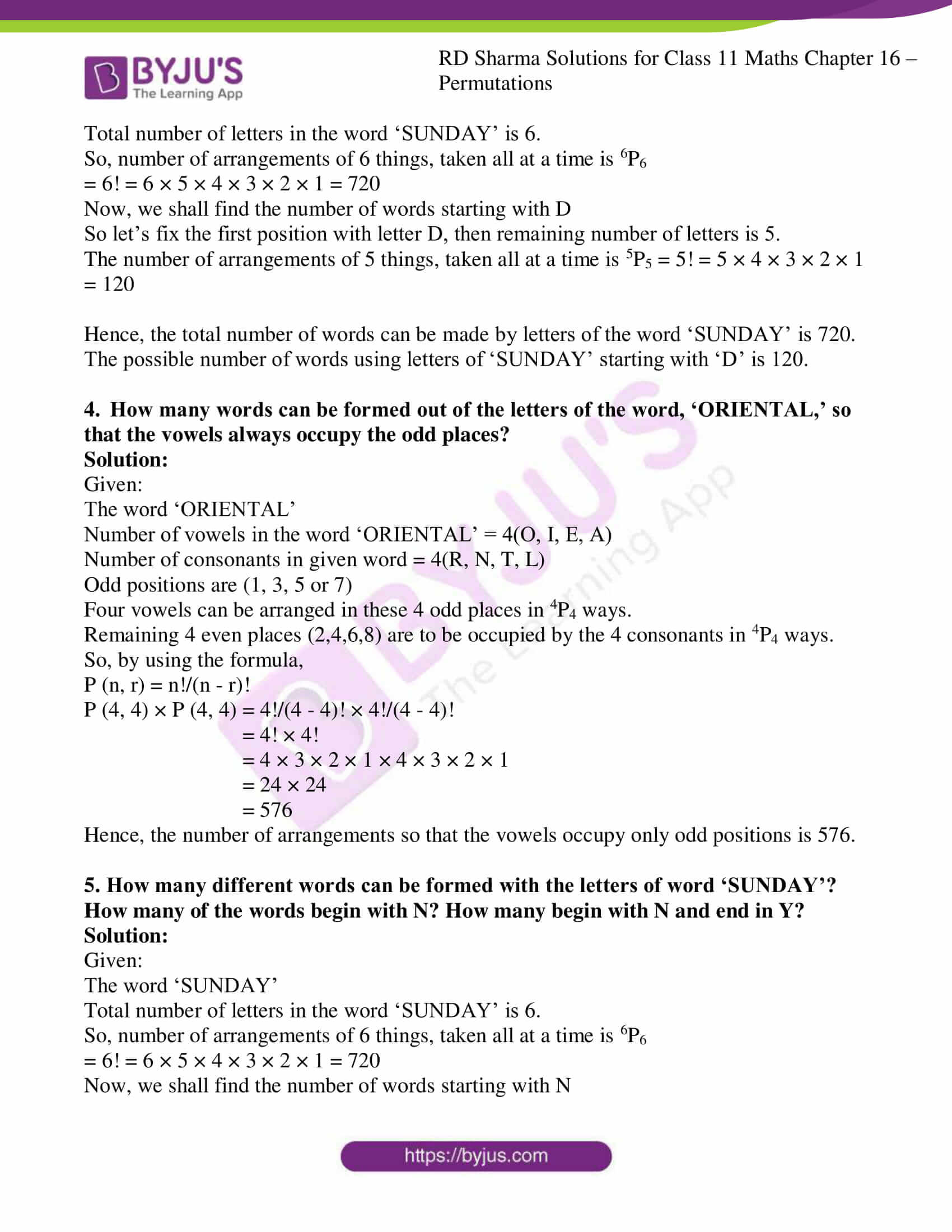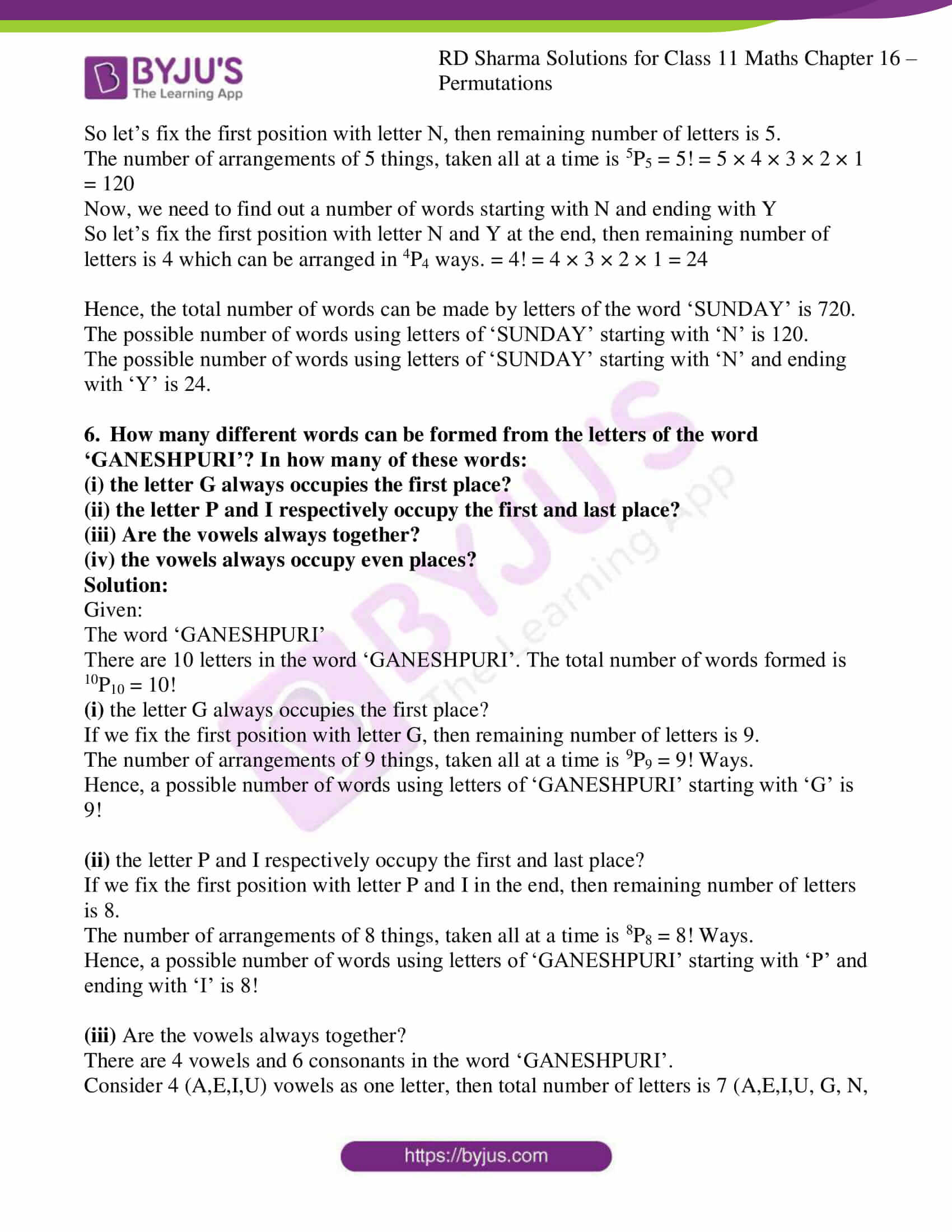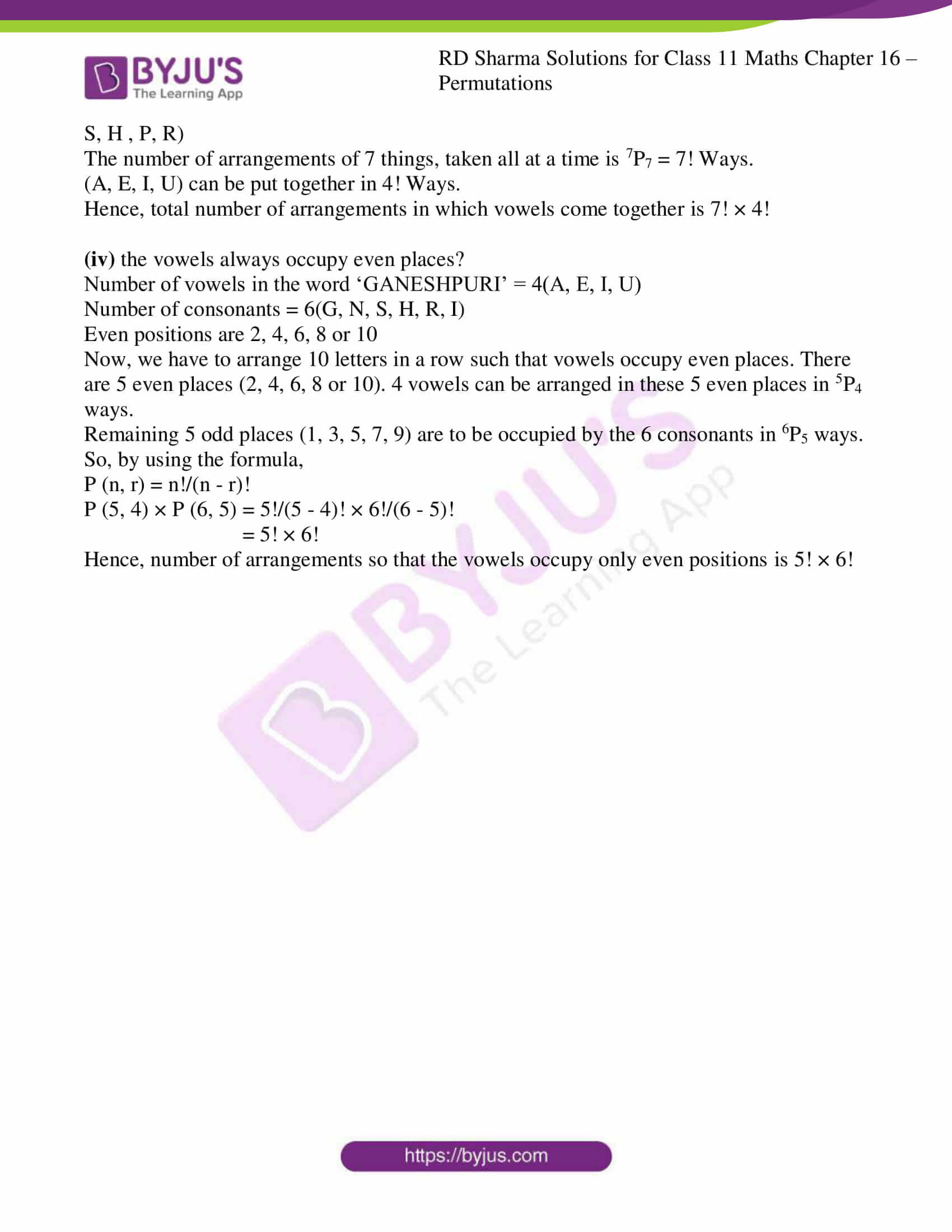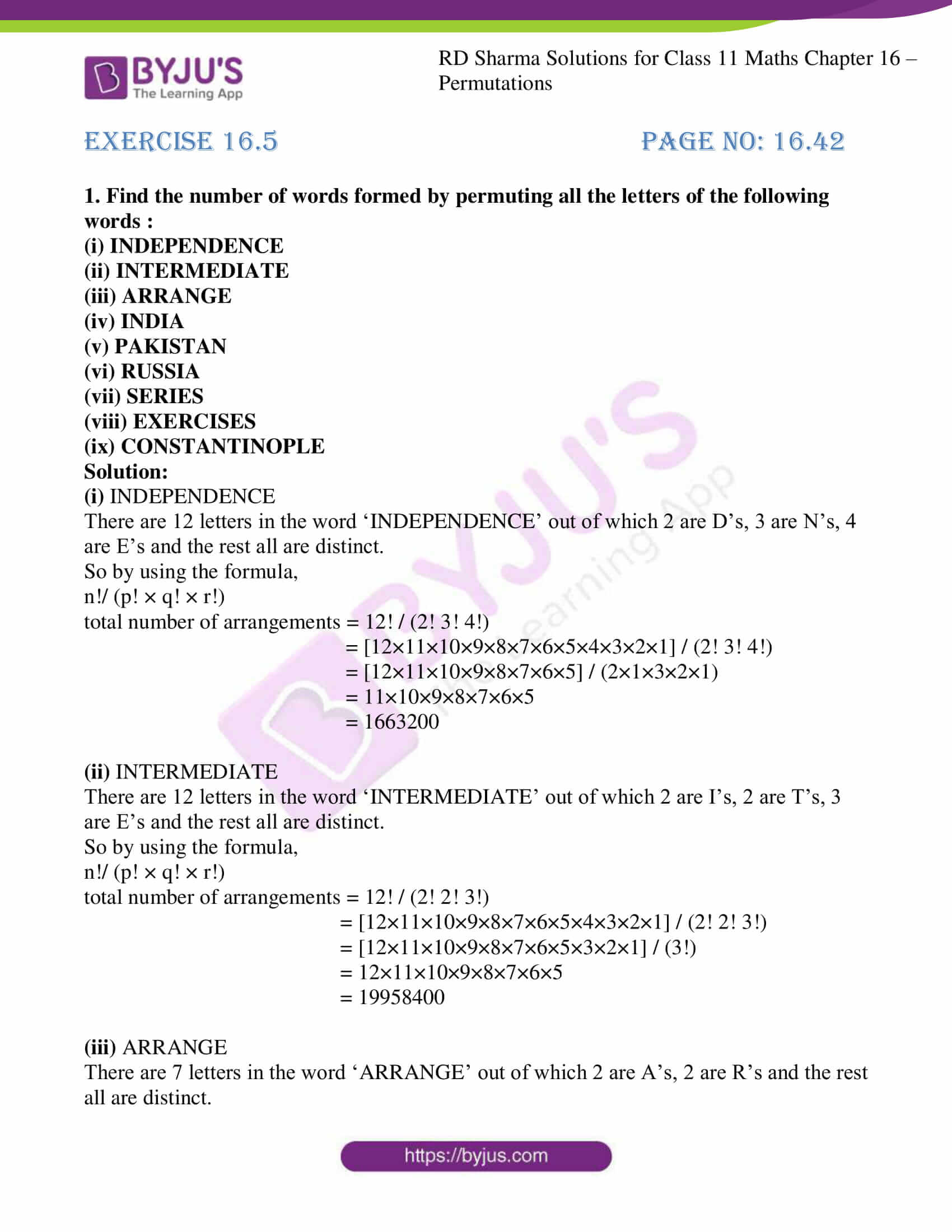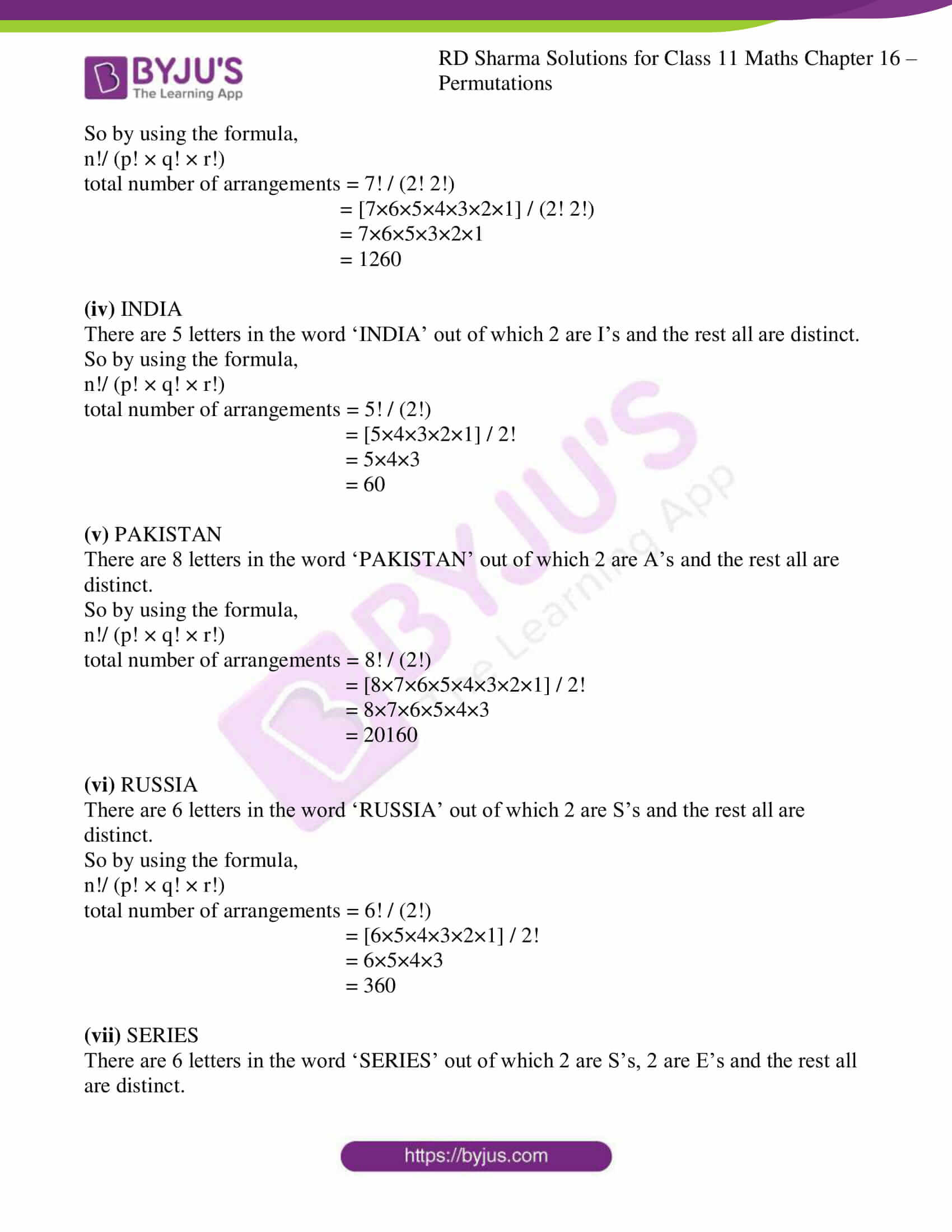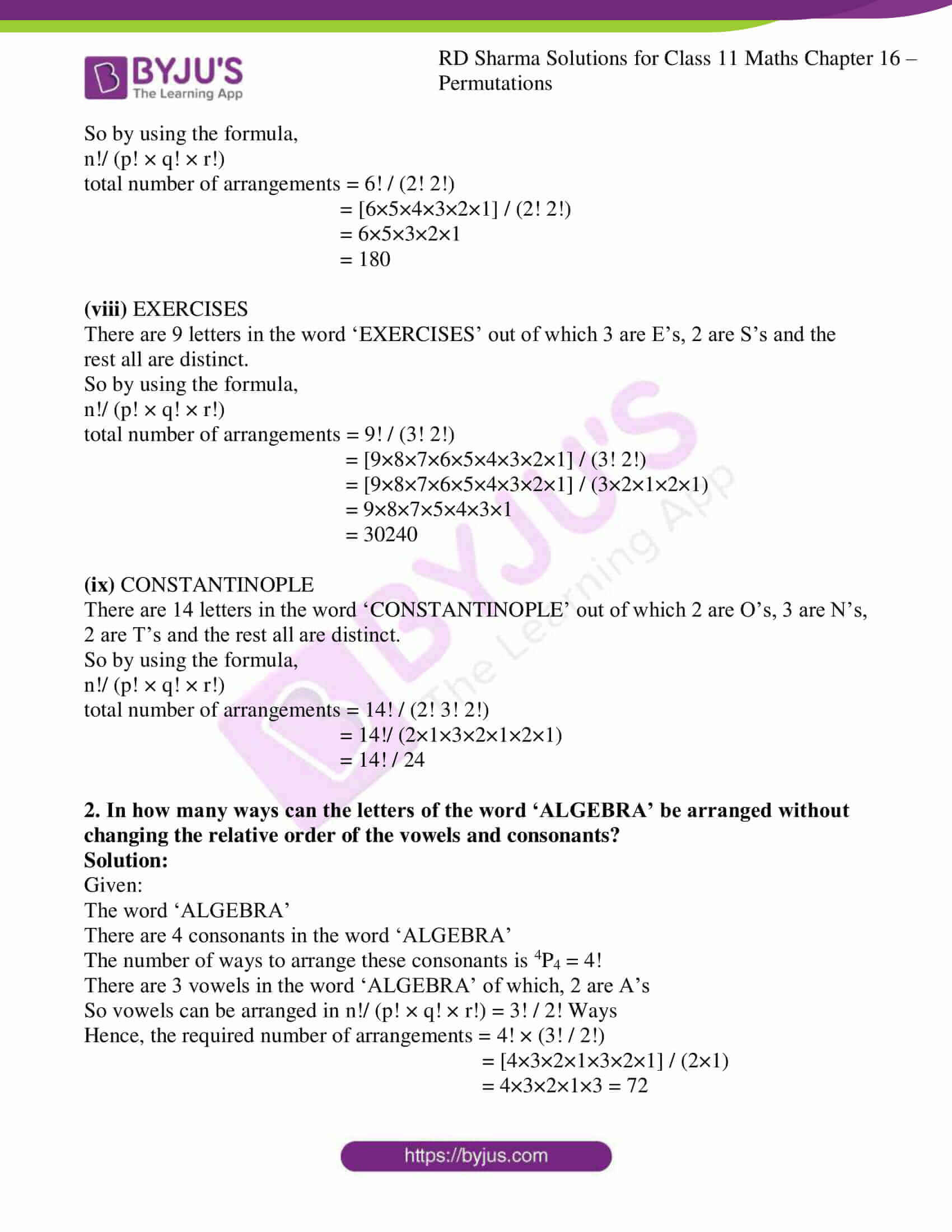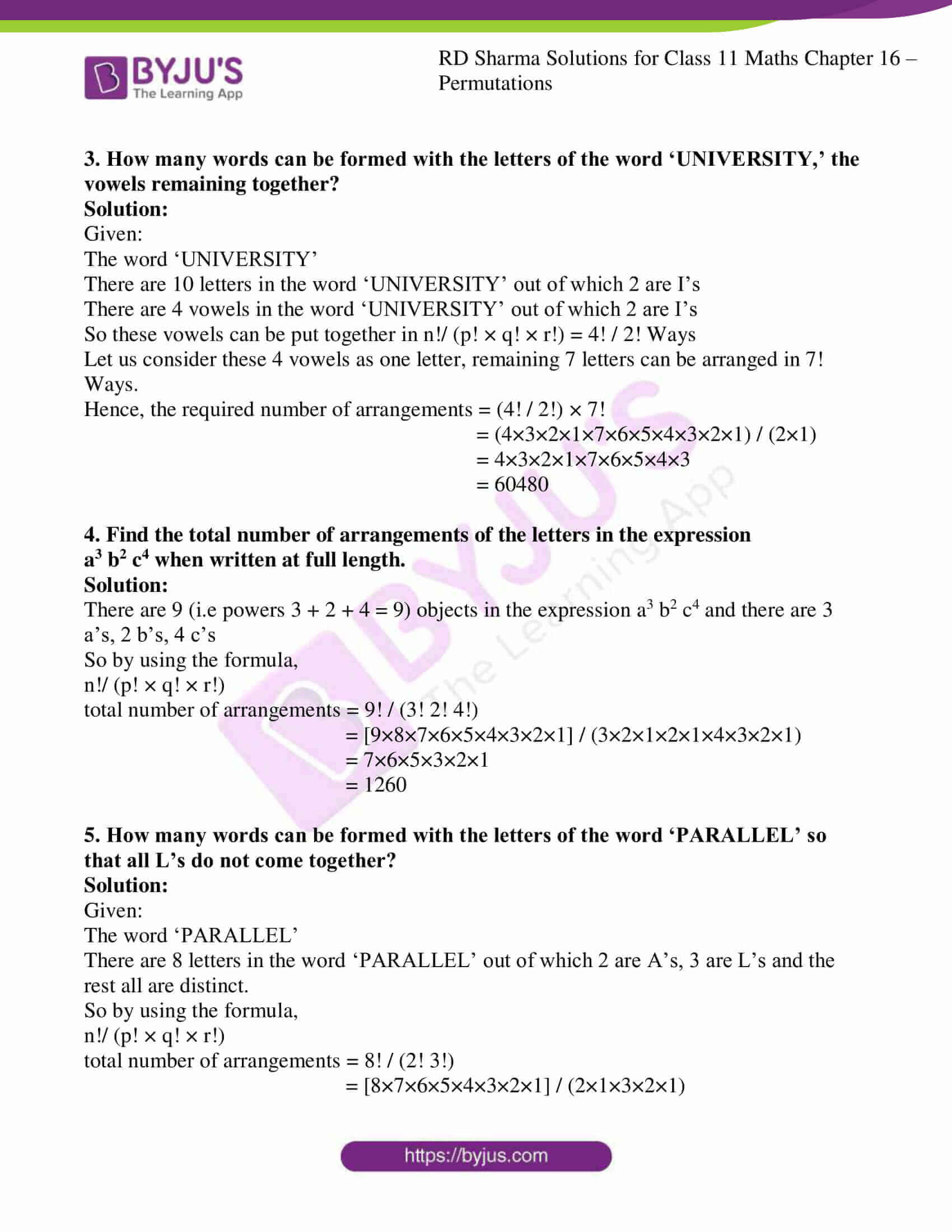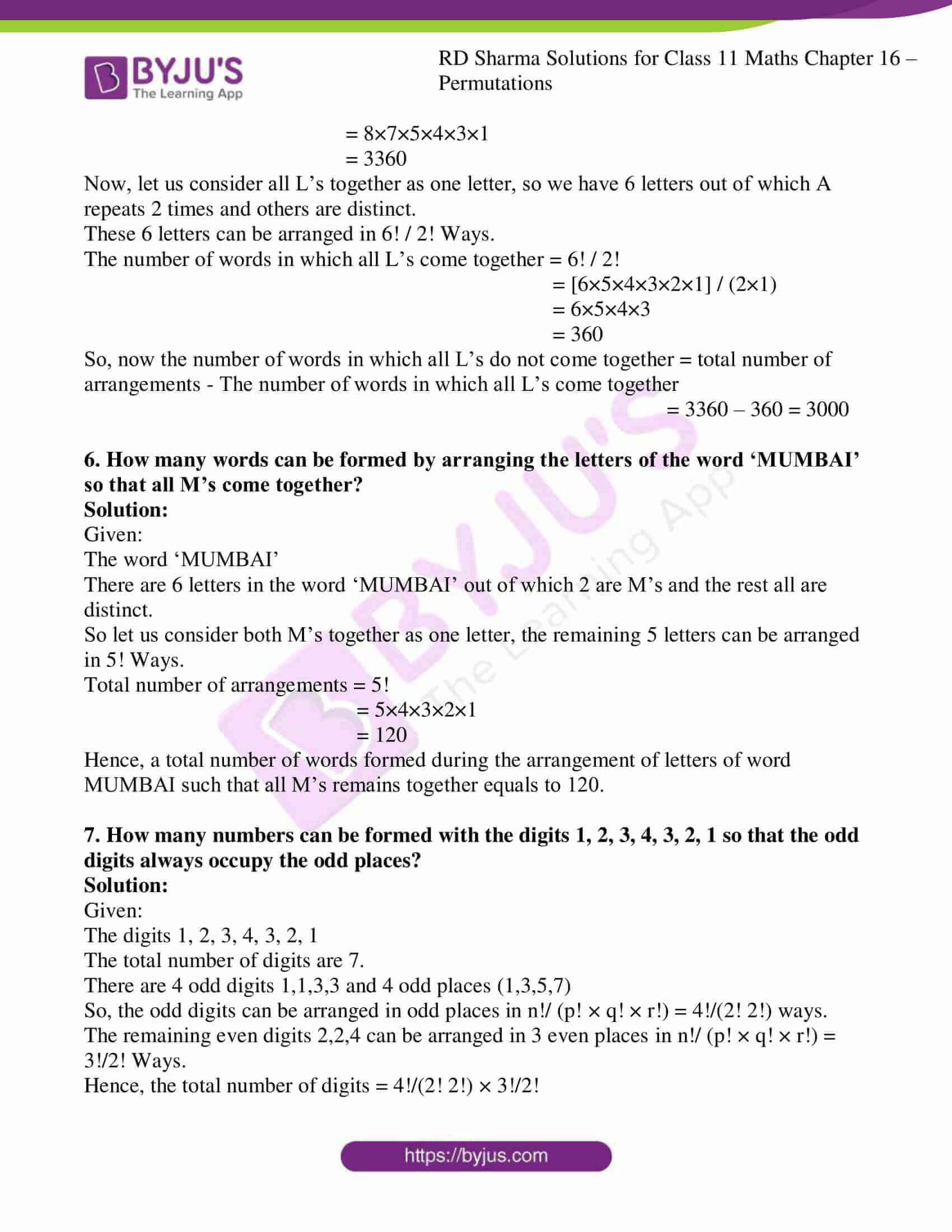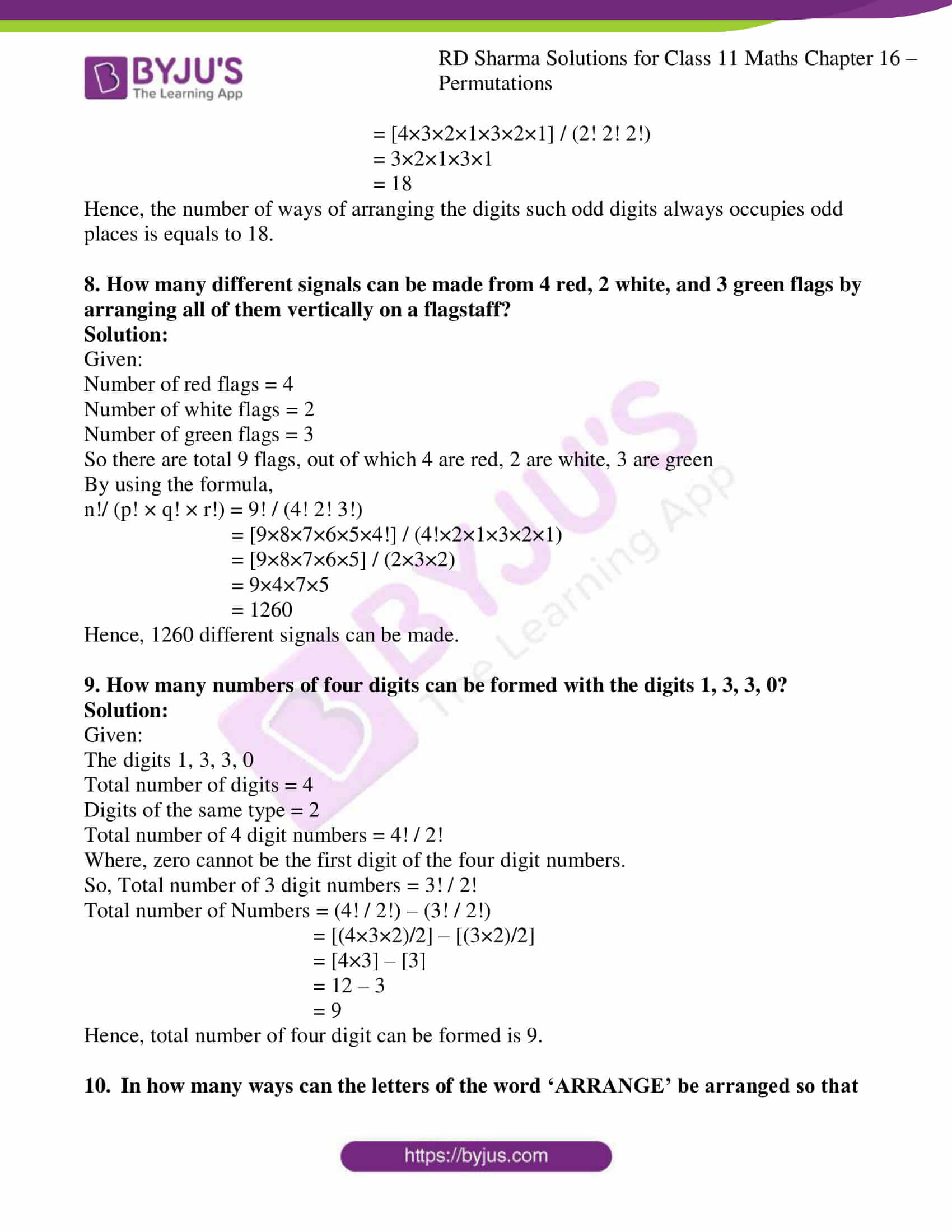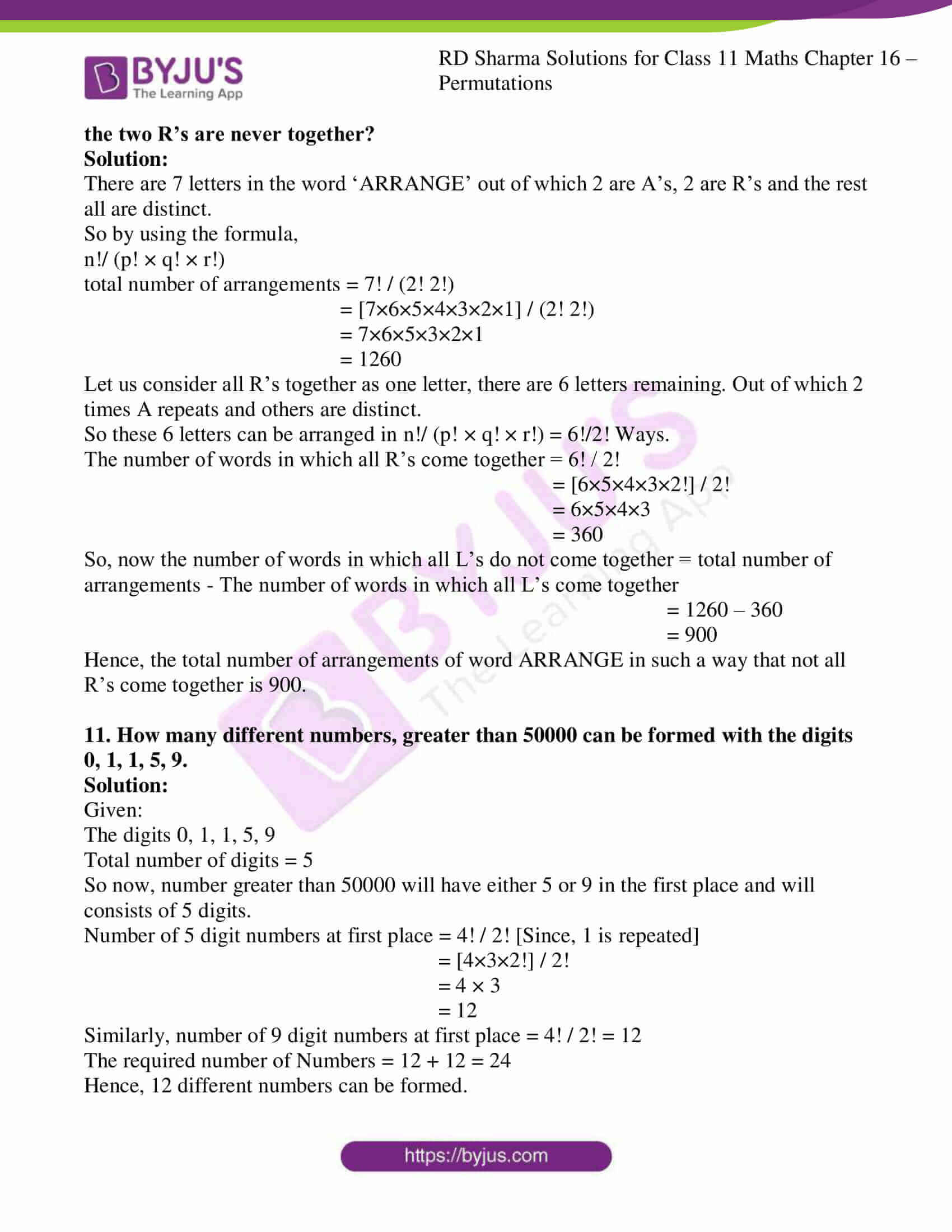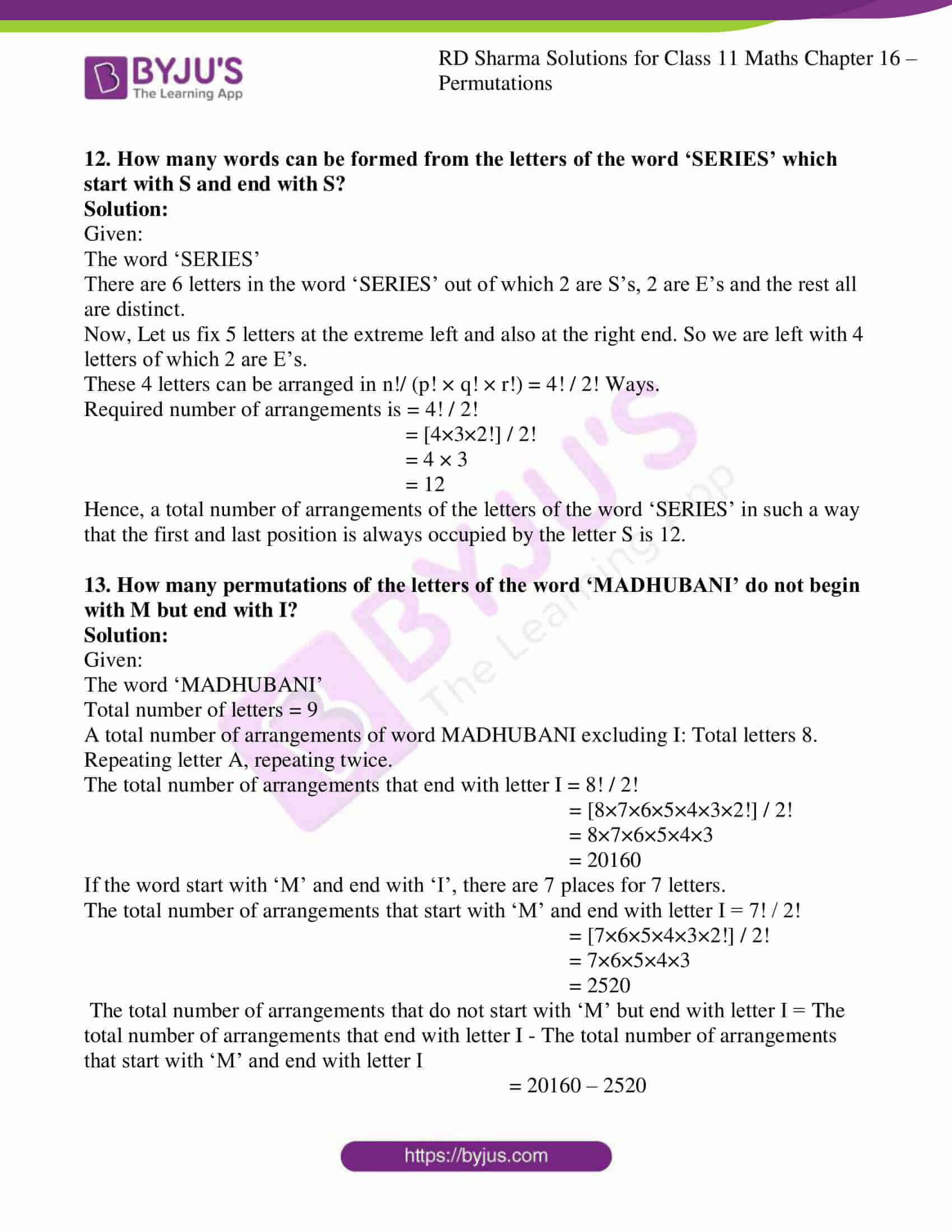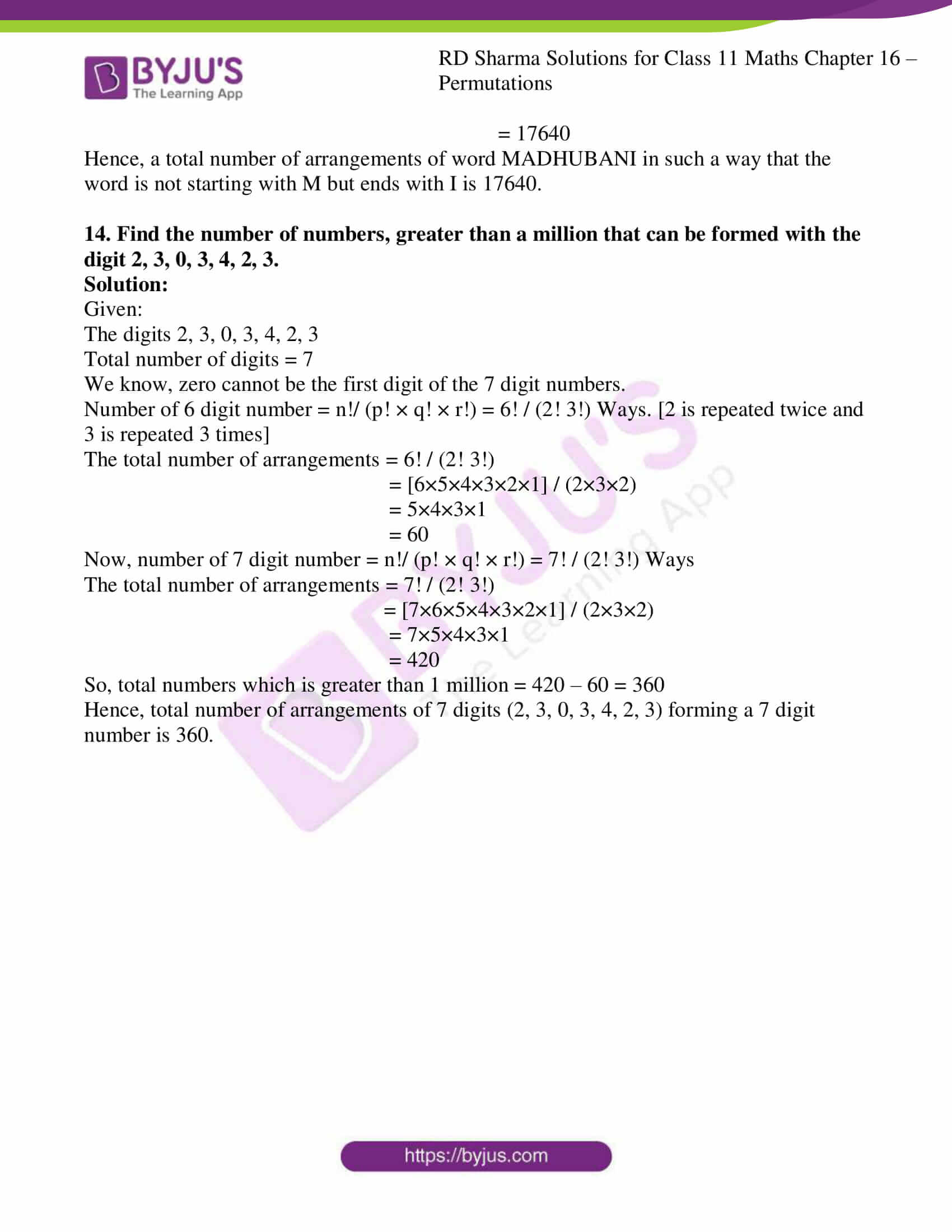### Access answers to RD Sharma Solutions for Class 11 Maths Chapter 16 – Permutations

EXERCISE 16.1 PAGE NO: 16.4

1. Compute:

(i) 30!/28!

(ii) (11! – 10!)/9!

(iii) L.C.M. (6!, 7!, 8!)

Solution:

(i) 30!/28!

Let us evaluate,

30!/28! = (30 × 29 × 28!)/28!

= 30 × 29

= 870

(ii) (11! – 10!)/9!

Let us evaluate,

We know,

11! = 11 × 10 × 9 × …. × 1

10! = 10 × 9 × 8 × … × 1

9! = 9 × 8 × 7 × … × 1

By using these values we get,

(11! – 10!)/9! = (11 × 10 × 9! – 10 × 9!)/ 9!

= 9! (110 – 10)/9!

= 110 – 10

= 100

(iii) L.C.M. (6!, 7!, 8!)

Let us find the LCM of (6!, 7!, 8!)

We know,

8! = 8 × 7 × 6!

7! = 7 × 6!

6! = 6!

So,

L.C.M. (6!, 7!, 8!) = LCM [8 × 7 × 6!, 7 × 6!, 6!]

= 8 × 7 × 6!

= 8!

2. Prove that: 1/9! + 1/10! + 1/11! = 122/11!

Solution:

Given:

1/9! + 1/10! + 1/11! = 122/11!

Let us consider LHS: 1/9! + 1/10! + 1/11!

1/9! + 1/10! + 1/11! = 1/9! + 1/(10×9!) + 1/(11×10×9!)

= (110 + 11 + 1)/(11 × 10 × 9!)

= 122/11!

= RHS

Hence proved.

3. Find x in each of the following:

(i) 1/4! + 1/5! = x/6!

(ii) x/10! = 1/8! + 1/9!

(iii) 1/6! + 1/7! = x/8!

Solution:

(i) 1/4! + 1/5! = x/6!

We know that

5! = 5 × 4 × 3 × 2 × 1

6! = 6 × 5 × 4 × 3 × 2 × 1

So by using these values,

1/4! + 1/5! = x/6!

1/4! + 1/(5×4!) = x/6!

(5 + 1) / (5×4!) = x/6!

6/5! = x/(6×5!)

x = (6 × 6 × 5!)/5!

= 36

∴ The value of x is 36.

(ii) x/10! = 1/8! + 1/9!

We know that

10! = 10 × 9!

9! = 9 × 8!

So by using these values,

x/10! = 1/8! + 1/9!

x/10! = 1/8! + 1/(9×8!)

x/10! = (9 + 1) / (9×8!)

x/10! = 10/9!

x/(10×9!) = 10/9!

x = (10 × 10 × 9!)/9!

= 10 × 10

= 100

∴ The value of x is 100.

(iii) 1/6! + 1/7! = x/8!

We know that

8! = 8 × 7 × 6!

7! = 7 × 6!

So by using these values,

1/6! + 1/7! = x/8!

1/6! + 1/(7×6!) = x/8!

(1 + 7)/(7×6!) = x/8!

8/7! = x/8!

8/7! = x/(8×7!)

x = (8 × 8 × 7!)/7!

= 8 × 8

= 64

∴ The value of x is 64.

4. Convert the following products into factorials:
(i) 5 ⋅ 6 ⋅ 7 ⋅ 8 ⋅ 9 ⋅ 10

(ii) 3 ⋅ 6 ⋅ 9 ⋅ 12 ⋅ 15 ⋅ 18

(iii) (n + 1) (n + 2) (n + 3) …(2n)
(iv) 1 ⋅ 3 ⋅ 5 ⋅ 7 ⋅ 9 … (2n – 1)

Solution:

(i) 5 ⋅ 6 ⋅ 7 ⋅ 8 ⋅ 9 ⋅ 10

Let us evaluate

We can write it as:

5 ⋅ 6 ⋅ 7 ⋅ 8 ⋅ 9 ⋅ 10 = (1×2×3×4×5×6×7×8×9×10)/(1×2×3×4)

= 10!/4!

(ii) 3 ⋅ 6 ⋅ 9 ⋅ 12 ⋅ 15 ⋅ 18

Let us evaluate

3 ⋅ 6 ⋅ 9 ⋅ 12 ⋅ 15 ⋅ 18 = (3×1) × (3×2) × (3×3) × (3×4) × (3×5) × (3×6)

= 36 (1×2×3×4×5×6)

= 36 (6!)

(iii) (n + 1) (n + 2) (n + 3) … (2n)

Let us evaluate

(n + 1) (n + 2) (n + 3) … (2n) = [(1) (2) (3) .. (n) … (n + 1) (n + 2) (n + 3) … (2n)] / (1) (2) (3) .. (n)

= (2n)!/n!

(iv) 1 ⋅ 3 ⋅ 5 ⋅ 7 ⋅ 9 … (2n – 1)

Let us evaluate

1 ⋅ 3 ⋅ 5 ⋅ 7 ⋅ 9 … (2n – 1) = [(1) (3) (5) … (2n-1)] [(2) (4) (6) … (2n)] / [(2) (4) (6) … (2n)]

= [(1) (2) (3) (4) … (2n-1) (2n)] / 2n [(1) (2) (3) … (n)]

= (2n)! / 2n n!

5. Which of the following are true:

(i) (2 + 3)! = 2! + 3!

(ii) (2 × 3)! = 2! × 3!

Solution:

(i) (2 + 3)! = 2! + 3!

Let us consider LHS: (2 + 3)!

(2 + 3)! = 5!

Now RHS,

2! + 3! = (2×1) + (3×2×1)

= 2 + 6

= 8

LHS ≠ RHS

∴ The given expression is false.

(ii) (2 × 3)! = 2! × 3!

Let us consider LHS: (2 × 3)!

(2 × 3)! = 6!

= 6 × 5 × 4 × 3 × 2 × 1

= 720

Now RHS,

2! × 3! = (2×1) × (3×2×1)

= 12

LHS ≠ RHS

∴ The given expression is false.

6. Prove that: n! (n + 2) = n! + (n + 1)!

Solution:

Given:

n! (n + 2) = n! + (n + 1)!

Let us consider RHS = n! + (n + 1)!

n! + (n + 1)! = n! + (n + 1) (n + 1 – 1)!

= n! + (n + 1)n!

= n!(1 + n + 1)

= n! (n + 2)

= L.H.S

L.H.S = R.H.S

Hence, Proved.

EXERCISE 16.2 PAGE NO: 16.14

1. In a class, there are 27 boys and 14 girls. The teacher wants to select 1 boy and 1 girl to represent the class in a function. In how many ways can the teacher make this selection?

Solution:

Given:

27 boys and 14 girls.

Here the teacher has to

(i) Select a boy among 27 boys, and

(ii) Select a girl among 14 girls.

Number of ways to select one boy is 27C1 and similarly the number of ways to select one girl is 14C1.

Hence, the number of ways to select 1 boy and 1 girl to represent the class in a function is

14C1 × 27C1 = 14 × 27 = 378 ways.

2. A person wants to buy one fountain pen, one ball pen, and one pencil from a stationery shop. If there are 10 fountain pen varieties, 12 ball pen varieties and 5 pencil varieties, in how many ways can he select these articles?

Solution:

Given:

10 fountain pens, 12 ball pens, and 5 pencil

Here the person has to

(i) Select a ball pen from 12 ball pens.

(ii) Select a fountain pen from 10 fountain pens, and

(iii) Select a pencil from 5 pencils.

The number of ways to select one fountain pen is 10C1 and similarly the number of ways to select one ball pen is 12C1 and number of ways to select one pencil from 5 pencils is 5C1

Hence, the number of ways to select one fountain pen, one ball pen and one pencil from a stationery shop is 10C1 × 12C1 × 5C1 = 10 × 12 × 5 = 600 ways.

3. From Goa to Bombay there are two roots; air, and sea. From Bombay to Delhi there are three routes; air, rail, and road. From Goa to Delhi via Bombay, how many kinds of routes are there?

Solution:

Given:

The number of roots from Goa to Bombay is air and sea.

So, the number of ways to go from Goa to Bombay is 2C1

Given: The number of roots from Bombay to Delhi are: air, rail, and road.

So, the number of ways to go from Bombay to Delhi is 3C1

Hence, the number of ways to go from Goa to Delhi via Bombay is 2C1 × 3C1 = 2 × 3 = 6 ways.

4. A mint prepares metallic calendars specifying months, dates and days in the form of monthly sheets (one plate for each month). How many types of calendars should it prepare to serve for all the possibilities in future years?

Solution:

The mint has to perform

(i) Select the number of days in the month of February (there can be 28 or 29 days), and

(ii) Select the first day of February.

Now,

In 2 ways mint can select the number of days in February and for selecting first day of February, it can start from any of one of the seven days of the week, so there are 7 possibilities.

Hence, the number of types calendars should it prepare to serve for all the possibilities in future years is 7 × 2 = 14.

5. There are four parcels and five post-offices. In how many different ways can the parcels be sent by registered post?

Solution:

Given:

Total number of parcels = 4

Total number of post-offices = 5

One parcel can be posted in 5 ways that is in either of the one post offices. So, 5C1. Similarly, for other parcels also it can be posted in 5C1 ways.

Hence the number of ways the parcels be sent by registered post is

5C1 × 5C1 × 5C1 × 5C1 = 5 × 5 × 5 × 5 = 625 ways.

6. A coin is tossed five times, and outcomes are recorded. How many possible outcomes are there?

Solution:

Given:

A coin is tossed 5 times, so each time the outcome is either heads or tails, so two possibilities are possible.

The total possible outcomes are:

2C1 × 2C1 × 2C1 × 2C1 × 2C1 = 2 × 2 × 2 × 2 × 2 = 32 outcomes.

7. In how many ways can an examinee answer a set of ten true/false type questions?

Solution:

Given:

An examinee can answer a question either true or false, so there are two possibilities.

The number of ways for an examinee to answer a set of ten true/false type questions are: 2C1 × 2C1 × 2C1 × 2C1 × 2C1 × 2C1 × 2C1 × 2C1 × 2C1 × 2C1 = 2×2×2×2×2×2×2×2×2×2 = 1024 ways

8. A letter lock consists of three rings each marked with 10 different letters. In how many ways it is possible to make an unsuccessful attempt to open the lock?

Solution:

The total number of ways to make an attempt to open the lock is = 10 × 10 × 10 = 1000

The number of successful attempts to open the lock = 1

The number of unsuccessful attempts to open the lock = 1000 – 1 = 999

Hence, required number of possible ways to make an unsuccessful attempt to open the lock is 999.

9. There are 6 multiple choice questions in an examination. How many sequences of answers are possible, if the first three questions have 4 choices each and the next three have 2 each?

Solution:

Given: Multiple choice question, only one answer is correct of the given options.

For the first three questions only one answer is correct out of four. So it can be answered in 4 ways.

Total number of ways to answer the first 3 questions = 4C1 × 4C1 × 4C1 = 4 × 4 × 4 = 64

Each of the next 3 questions can be answered in 2 ways.

Total number of ways to answer the next 3 questions = 2C1 × 2C1 × 2C1 = 2 × 2 × 2 = 8

Hence, total possible outcomes possible are 64 × 8= 512

10. There are 5 books on Mathematics and 6 books on Physics in a book shop. In how many ways can a student buy:
(i) a Mathematics book and a Physics book
(ii) either a Mathematics book or a Physics book?

Solution:

(i) Given: there are 5 books of mathematics and 6 books of physics.

In order to buy one mathematics book, number of ways is 5C1 similarly to buy one physics book number of ways is 6C1

Hence, the number of ways a student buy a Mathematics book and a Physics book is 5C1 × 6C1 = 5 × 6 = 30

(ii) Given: there is a total of 11 books.

So in order to buy either a Mathematics book or a Physics book it means that only one book out of eleven is bought.

Hence, the number of ways in which a student can either buy either a Mathematics book or a Physics book is 11C1 = 11

11. Given 7 flags of different colours, how many different signals can be generated if a signal requires the use of two flags, one below the other?

Solution:

Given: seven flags are available and out of which two are needed to make a signal.

From this, we can say, that we have to select two flags out of seven and arrange these two flags to get one signal.

Seven flags of different colours are available, so first flag can be selected in 7 ways.

Now, the second flag can be selected from any one of the remaining flags in 6 ways.

Hence, the required number of signals are 7 × 6 = 42.

12. A team consists of 6 boys and 4 girls, and other has 5 boys and 3 girls. How many single matches can be arranged between the two teams when a boy plays against a boy, and a girl plays against a girl?

Solution:

Given:

A team consists of 6 boys and 4 girls, and other team has 5 boys and 3 girls.

Let team 1 be = 6 boys and 4 girls

Team 2 be = 5 boys and 3 girls

Singles matches are to be played, either a boy plays against a boy, and a girl plays against a girl.

So, number of ways to select a boy from team 1 is 6C1. Similarly, Number of ways to select a boy from team 2 is 5C1

Hence number of singles matches between boys is 6C1 × 5C1 = 6 × 5 = 30

A number of ways to select a girl from team 1 is 4C1. Similarly, Number of ways to select a girl from team 2 is 3C1

Hence number of singles matches between girls is 3C1 × 4C1 = 4 × 3 = 12

∴ The total number of single matches are = 30 + 12 = 42

13. Twelve students compete in a race. In how many ways first three prizes be given?

Solution:

Given:

Twelve students compete in a race.

Number of ways to select the winner of the first prize is 12C1

Number of ways to select the winner of the second prize is 11C1 (11, since one student is already given a prize)

Number of ways to select the winner of the third prize is 10C1 (10, since two students are already given a prize)

Hence, total number of ways is 12C1 × 11C1 × 10C1 = 12 × 11 × 10 = 1320.

14. How many A.P.’s with 10 terms are there whose first term is in the set {1, 2, 3} and whose common difference is in the set {1, 2, 3, 4, 5}?

Solution:

We know that, each AP consists of a unique first term and a common difference.

So, number of ways to select the first term of a given set is 3C1 = 3

And, number of ways to select a common difference of given set is 5C1 = 5

Hence, total number of AP’s possible are 3C1 × 5C1 = 3 × 5 = 15

15. From among the 36 teachers in a college, one principal, one vice-principal and the teacher-in-charge are to be appointed. In how many ways can this be done?

Solution:

Number of ways to appoint one principal, vice-principal and the teacher incharge is equal to the number of ways to select the three teachers from 36 members.

So, a total of three positions are to be appointed.

Number of ways to select principal is 36C1 = 36

Number of ways to select vice-principal is 35C1 = 35 (35, since one position is already given)

Number of ways to select teacher in charge is 34C1 (34, since two positions are already given)

Hence, number of ways to appoint three teachers is 36C1 × 35C1 × 34C1 = 36 × 35 × 34 = 42840.

16. How many three-digit numbers are there with no digit repeated?

Solution:

Let us assume we have three boxes.

The first box can be filled with any one of the nine digits (0 not allowed at first place).

So, the possibilities are 9C1

The second box can be filled with any one of the nine digits

So the available possibilities are 9C1

The third box can be filled with any one of the eight digits

So the available possibilities are 8C1

Hence, the total number of possible outcomes are 9C1 × 9C1 × 8C1 = 9 × 9 × 8 = 648.

17. How many three-digit numbers are there?

Solution:

Let us assume we have three boxes.

The first box can be filled with any one of the nine digits (zero not allowed at first position)

So, possibilities are 9C1

The second box can be filled with any one of the ten digits

So the available possibilities are 10C1

Third box can be filled with any one of the ten digits

So the available possibilities are 10C1

Hence, the total number of possible outcomes are 9C1 × 10C1 × 10C1 = 9 × 10 × 10 = 900.

18. How many three-digit odd numbers are there?

Solution:

We know that in odd numbers, the last digit consists of (1, 3, 5, 7, 9).

Let us assume we have three boxes.

The first box can be filled with any one of the nine digits (zero not allowed at first position)

So the possibilities are 9C1

The second box can be filled with any one of the ten digits

So the available possibilities are 10C1

The third box can be filled with any one of the five digits (1,3,5,7,9)

So the available possibilities are 5C1

Hence, the total number of possible outcomes are 9C1 × 10C1 × 5C1 = 9 × 10 × 5 = 450

19. How many different five-digit number license plates can be made if
(i) the first digit cannot be zero, and the repetition of digits is not allowed,
(ii) the first-digit cannot be zero, but the repetition of digits is allowed?

Solution:

(i) We know that zero cannot be the first digit of the license plates. And the repetition of digits is not allowed.

Let us assume five boxes, now the first box can be filled with one of the nine available digits, so the possibility is 9C1

Similarly, the second box can be filled with one of the nine available digits, so the possibility is 9C1

The third box can be filled with one of the eight available digits, so the possibility is 8C1

The fourth box can be filled with one of the seven available digits, so the possibility is 7C1

The fifth box can be filled with one of the six available digits, so the possibility is 6C1

Hence, the total number of possible outcomes is 9C1 × 9C1 × 8C1 × 7C1 × 6C1 = 9 × 9 × 8 × 7 × 6 = 27,216

(ii) We know that zero cannot be the first digit of the license plates. And the repetition of digits is allowed.

Let us assume five boxes, now the first box can be filled with one of the nine available digits, so the possibility is 9C1

Similarly, the second box can be filled with one of the ten available digits, so the possibility is 10C1

The third box can be filled with one of the ten available digits, so the possibility is 10C1

The fourth box can be filled with one of the ten available digits, so the possibility is 10C1

The fifth box can be filled with one of the ten available digits, so the possibility is 10C1

Hence, the total number of possible outcomes is  9C1 × 10C1 × 10C1 × 10C1 × 10C1 = 9 × 10 × 10 × 10 × 10 = 90,000

20. How many four-digit numbers can be formed with the digits 3, 5, 7, 8, 9 which are greater than 7000, if repetition of digits is not allowed?

Solution:

The required numbers are greater than 7000.

So, the thousand’s place can be filled with any of the 3 digits: 7, 8, 9.

Let us assume four boxes, now in the first box can either be one of the three numbers 7, 8 or 9, so there are three possibilities which are 3C1

In the second box, the numbers can be any of the four digits left, so the possibility is 4C1

In the third box, the numbers can be any of the three digits left, so the possibility is 3C1

In the fourth box, the numbers can be any of the two digits left, so the possibility is 2C1

Hence, the total number of possible outcomes is 3C1 × 4C1 × 3C1 × 2C1 = 3 × 4 × 3 × 2 = 72.

21. How many four-digit numbers can be formed with the digits 3, 5, 7, 8, 9 which are greater than 8000, if repetition of digits is not allowed?

Solution:

The required numbers are greater than 8000.

So, the thousand’s place can be filled with 2 digits: 8 or 9.

Let us assume four boxes, now in the first box can either be one of the two numbers 8 or 9, so there are two possibilities which is 2C1

In the second box, the numbers can be any of the four digits left, so the possibility is 4C1

In the third box, the numbers can be any of the three digits left, so the possibility is 3C1

In the fourth box, the numbers can be any of the two digits left, so the possibility is 2C1

Hence total number of possible outcomes is 2C1 × 4C1 × 3C1 × 2C1 = 2 × 4 × 3 × 2 = 48.

22. In how many ways can six persons be seated in a row?

Solution:

Let us assume there are six seats.

In the first seat, any one of six members can be seated, so the total number of possibilities is 6C1

In the second seat, any one of five members can be seated, so the total number of possibilities is 5C1 ways.

In the third seat, any one of four members can be seated, so the total number of possibilities is 4C1 ways.

In the fourth seat, any one of three members can be seated, so the total number of possibilities is 3C1 ways.

In the fifth seat, any one of two members can be seated, so the total number of possibilities is 2C1 ways.

In the sixth seat, only one remaining person can be seated, so the total number of possibilities is 1C1 ways.

Hence, the total number of possible outcomes = 6C1 × 5C1 × 4C1 × 3C1 × 2C1 × 1C1 = 6 × 5 × 4 × 3 × 2 × 1 = 720.

23. How many 9-digit numbers of different digits can be formed?

Solution:

In a nine-digit number, 0 cannot appear in the first digit and the repetition of digits is not allowed. So, the number of ways of filling up the first digit is 9C1= 9

Now, 9 digits are left including 0. So, the second digit can be filled with any of the remaining 9 digits in 9 ways.

Similarly, the third box can be filled with one of the eight available digits, so the possibility is 8C1

The fourth digit can be filled with one of the seven available digits, so the possibility is 7C1

The fifth digit can be filled with one of the six available digits, so the possibility is 6C1

The sixth digit can be filled with one of the six available digits, so the possibility is 5C1

The seventh digit can be filled with one of the six available digits, so the possibility is 4C1

The eighth digit can be filled with one of the six available digits, so the possibility is 3C1

The ninth digit can be filled with one of the six available digits, so the possibility is 2 C1

Hence the number of total possible outcomes is 9C1 × 9C1 × 8C1 × 7C1 × 6C1 = 9 × 9 × 8 × 7 × 6 × 5 × 4 × 3 × 2 = 9 (9!)

24. How many odd numbers less than 1000 can be formed by using the digits 0, 3, 5, 7 when repetition of digits is not allowed?

Solution:

Any number less than 1000 may be any of a number from one-digit number, two-digit number and three-digit number.

Case 1: One-digit odd number

In order to make the number odd, the last digit has to be either of (3, 5, 7)

In the first box either of the three digits (3,5,7) can be placed, so the possibility is 3C1 = 3 possible ways.

Case 2: two-digit odd number

Tens place can be filled up by 3 ways (using any of the digit among 3, 5 and 7) and then the ones place can be filled in any of the remaining 2 digits.

So, there are 3 × 2 = 6 such 2-digit numbers

Case 3: three-digit odd number

Ignore zero at one’s place for some instance.

Hundreds place can be filled up in 3 ways (using any of any of the digit among 3, 5 and 7), then tens place in 3 ways by using remaining 3 digits (after using a digit, there will be three digits) and then the ones place in 2 ways.

So, there are a total of 3 *3 * 2 = 18 numbers of 3-digit numbers which includes both odd and even numbers (ones place digit are zero). In order to get the odd numbers, it is required to ignore the even numbers i.e. numbers ending with zero.

To obtain the even 3-digit numbers, one’s place can be filled up in 1 way (only 0 to be filled), hundreds place in 3 ways (using any of the digit among 3, 5, 7) and then tens place in 2 ways (using remaining 2 digits after filling up hundreds place).

So, there are a total of 1 * 3 * 2 = 6 even 3-digit numbers using the digits 0, 3, 5 and 7 (repetition not allowed)

Then, number of three-digit odd numbers using the digits 0, 3, 5 and 7 (repetition not allowed) = 18 –  6 = 12.

∴ The odd numbers less than 1000 that can be formed by using the digits 0, 3, 5, 7 when repetition of digits is not allowed are 3 + 6 + 12 = 21.

EXERCISE 16.3 PAGE NO: 16.28

1. Evaluate each of the following:

(i) 8P3

(ii) 10P4

(iii) 6P6

(iv) P (6, 4)

Solution:

(i) 8P3

We know that, 8P3 can be written as P (8, 3)

By using the formula,

P (n, r) = n!/(n – r)!

P (8, 3) = 8!/(8 – 3)!

= 8!/5!

= (8 × 7 × 6 × 5!)/5!

= 8 × 7 × 6

= 336

8P3 = 336

(ii) 10P4

We know that, 10P4 can be written as P (10, 4)

By using the formula,

P (n, r) = n!/(n – r)!

P (10, 4) = 10!/(10 – 4)!

= 10!/6!

= (10 × 9 × 8 × 7 × 6!)/6!

= 10 × 9 × 8 × 7

= 5040

10P4 = 5040

(iii) 6P6

We know that, 6P6 can be written as P (6, 6)

By using the formula,

P (n, r) = n!/(n – r)!

P (6, 6) = 6!/(6 – 6)!

= 6!/0!

= (6 × 5 × 4 × 3 × 2 × 1)/1 [Since, 0! = 1]

= 6 × 5 × 4 × 3 × 2 × 1

= 720

6P6 = 720

(iv) P (6, 4)

By using the formula,

P (n, r) = n!/(n – r)!

P (6, 4) = 6!/(6 – 4)!

= 6!/2!

= (6 × 5 × 4 × 3 × 2!)/2!

= 6 × 5 × 4 × 3

= 360

∴ P (6, 4) = 360

2. If P (5, r) = P (6, r – 1), find r.

Solution:

Given:

P (5, r) = P (6, r – 1)

By using the formula,

P (n, r) = n!/(n – r)!

P (5, r) = 5!/(5 – r)!

P (6, r-1) = 6!/(6 – (r-1))!

= 6!/(6 – r + 1)!

= 6!/(7 – r)!

So, from the question,

P (5, r) = P (6, r – 1)

Substituting the obtained values in above expression we get,

5!/(5 – r)! = 6!/(7 – r)!

Upon evaluating,

(7 – r)! / (5 – r)! = 6!/5!

[(7 – r) (7 – r – 1) (7 – r – 2)!] / (5 – r)! = (6 × 5!)/5!

[(7 – r) (6 – r) (5 – r)!] / (5 – r)! = 6

(7 – r) (6 – r) = 6

42 – 6r – 7r + r2 = 6

42 – 6 – 13r + r2 = 0

r2 – 13r + 36 = 0

r2 – 9r – 4r + 36 = 0

r(r – 9) – 4(r – 9) = 0

(r – 9) (r – 4) = 0

r = 9 or 4

For, P (n, r): r ≤ n

∴ r = 4 [for, P (5, r)]

3. If 5 P(4, n) = 6 P(5, n – 1), find n.

Solution:

Given:

5 P(4, n) = 6 P(5, n – 1)

By using the formula,

P (n, r) = n!/(n – r)!

P (4, n) = 4!/(4 – n)!

P (5, n-1) = 5!/(5 – (n-1))!

= 5!/(5 – n + 1)!

= 5!/(6 – n)!

So, from the question,

5 P(4, n) = 6 P(5, n – 1)

Substituting the obtained values in above expression we get,

5 × 4!/(4 – n)! = 6 × 5!/(6 – n)!

Upon evaluating,

(6 – n)! / (4 – n)! = 6/5 × 5!/4!

[(6 – n) (6 – n – 1) (6 – n – 2)!] / (4 – n)! = (6 × 5 × 4!) / (5 × 4!)

[(6 – n) (5 – n) (4 – n)!] / (4 – n)! = 6

(6 – n) (5 – n) = 6

30 – 6n – 5n + n2 = 6

30 – 6 – 11n + n2 = 0

n2 – 11n + 24 = 0

n2 – 8n – 3n + 24 = 0

n(n – 8) – 3(n – 8) = 0

(n – 8) (n – 3) = 0

n = 8 or 3

For, P (n, r): r ≤ n

∴ n = 3 [for, P (4, n)]

4. If P(n, 5) = 20 P(n, 3), find n.

Solution:

Given:

P(n, 5) = 20 P(n, 3)

By using the formula,

P (n, r) = n!/(n – r)!

P (n, 5) = n!/(n – 5)!

P (n, 3) = n!/(n – 3)!

So, from the question,

P(n, 5) = 20 P(n, 3)

Substituting the obtained values in above expression we get,

n!/(n – 5)! = 20 × n!/(n – 3)!

Upon evaluating,

n! (n – 3)! / n! (n – 5)! = 20

[(n – 3) (n – 3 – 1) (n – 3 – 2)!] / (n – 5)! = 20

[(n – 3) (n – 4) (n – 5)!] / (n – 5)! = 20

(n – 3) (n – 4) = 20

n2 – 3n – 4n + 12 = 20

n2 – 7n + 12 – 20 = 0

n2 – 7n – 8 = 0

n2 – 8n + n – 8 = 0

n(n – 8) – 1(n – 8) = 0

(n – 8) (n – 1) = 0

n = 8 or 1

For, P(n, r): n ≥ r

∴ n = 8 [for, P(n, 5)]

5. If nP4 = 360, find the value of n.

Solution:

Given:

nP4 = 360

nP4 can be written as P (n , 4)

By using the formula,

P (n, r) = n!/(n – r)!

P (n, 4) = n!/(n – 4)!

So, from the question,

nP4 = P (n, 4) = 360

Substituting the obtained values in above expression we get,

n!/(n – 4)! = 360

[n(n – 1) (n – 2) (n – 3) (n – 4)!] / (n – 4)! = 360

n (n – 1) (n – 2) (n – 3) = 360

n (n – 1) (n – 2) (n – 3) = 6×5×4×3

On comparing,

The value of n is 6.

6. If P(9, r) = 3024, find r.

Solution:

Given:

P (9, r) = 3024

By using the formula,

P (n, r) = n!/(n – r)!

P (9, r) = 9!/(9 – r)!

So, from the question,

P (9, r) = 3024

Substituting the obtained values in above expression we get,

9!/(9 – r)! = 3024

1/(9 – r)! = 3024/9!

= 3024/(9×8×7×6×5×4×3×2×1)

= 3024/(3024×5×4×3×2×1)

= 1/5!

(9 – r)! = 5!

9 – r = 5

-r = 5 – 9

-r = -4

∴ The value of r is 4.

7. If P (11, r) = P (12, r – 1), find r.

Solution:

Given:

P (11, r) = P (12, r – 1)

By using the formula,

P (n, r) = n!/(n – r)!

P (11, r) = 11!/(11 – r)!

P (12, r-1) = 12!/(12 – (r-1))!

= 12!/(12 – r + 1)!

= 12!/(13 – r)!

So, from the question,

P (11, r) = P (12, r – 1)

Substituting the obtained values in above expression we get,

11!/(11 – r)! = 12!/(13 – r)!

Upon evaluating,

(13 – r)! / (11 – r)! = 12!/11!

[(13 – r) (13 – r – 1) (13 – r – 2)!] / (11 – r)! = (12×11!)/11!

[(13 – r) (12 – r) (11 -r)!] / (11 – r)! = 12

(13 – r) (12 – r) = 12

156 – 12r – 13r + r2 = 12

156 – 12 – 25r + r2 = 0

r2 – 25r + 144 = 0

r2 – 16r – 9r + 144 = 0

r(r – 16) – 9(r – 16) = 0

(r – 9) (r – 16) = 0

r = 9 or 16

For, P (n, r): r ≤ n

∴ r = 9 [for, P (11, r)]

8. If P(n, 4) = 12. P(n, 2), find n.

Solution:

Given:

P (n, 4) = 12. P (n, 2)

By using the formula,

P (n, r) = n!/(n – r)!

P (n, 4) = n!/(n – 4)!

P (n, 2) = n!/(n – 2)!

So, from the question,

P (n, 4) = 12. P (n, 2)

Substituting the obtained values in above expression we get,

n!/(n – 4)! = 12 × n!/(n – 2)!

Upon evaluating,

n! (n – 2)! / n! (n – 4)! = 12

[(n – 2) (n – 2 -1) (n – 2 – 2)!] / (n – 4)! = 12

[(n – 2) (n – 3) (n – 4)!] / (n – 4)! = 12

(n – 2) (n – 3) = 12

n2 – 3n – 2n + 6 = 12

n2 – 5n + 6 – 12 = 0

n2 – 5n – 6 = 0

n2 – 6n + n – 6 = 0

n (n – 6) – 1(n – 6) = 0

(n – 6) (n – 1) = 0

n = 6 or 1

For, P (n, r): n ≥ r

∴ n = 6 [for, P (n, 4)]

9. If P(n – 1, 3) : P(n, 4) = 1 : 9, find n.

Solution:

Given:

P (n – 1, 3): P (n, 4) = 1 : 9

P (n – 1, 3)/ P (n, 4) = 1 / 9

By using the formula,

P (n, r) = n!/(n – r)!

P (n – 1, 3) = (n – 1)! / (n – 1 – 3)!

= (n – 1)! / (n – 4)!

P (n, 4) = n!/(n – 4)!

So, from the question,

P (n – 1, 3)/ P (n, 4) = 1 / 9

Substituting the obtained values in above expression we get,

[(n – 1)! / (n – 4)!] / [n!/(n – 4)!] = 1/9

[(n – 1)! / (n – 4)!] × [(n – 4)! / n!] = 1/9

(n – 1)!/n! = 1/9

(n – 1)!/n (n – 1)! = 1/9

1/n = 1/9

n = 9

∴ The value of n is 9.

10. If P(2n – 1, n) : P(2n + 1, n – 1) = 22 : 7 find n.

Solution:

Given:

P(2n – 1, n) : P(2n + 1, n – 1) = 22 : 7

P(2n – 1, n) / P(2n + 1, n – 1) = 22 / 7

By using the formula,

P (n, r) = n!/(n – r)!

P (2n – 1, n) = (2n – 1)! / (2n – 1 – n)!

= (2n – 1)! / (n – 1)!

P (2n + 1, n – 1) = (2n + 1)! / (2n + 1 – n + 1)!

= (2n + 1)! / (n + 2)!

So, from the question,

P(2n – 1, n) / P(2n + 1, n – 1) = 22 / 7

Substituting the obtained values in above expression we get,

[(2n – 1)! / (n – 1)!] / [(2n + 1)! / (n + 2)!] = 22/7

[(2n – 1)! / (n – 1)!] × [(n + 2)! / (2n + 1)!] = 22/7

[(2n – 1)! / (n – 1)!] × [(n + 2) (n + 2 – 1) (n + 2 – 2) (n + 2 – 3)!] / [(2n + 1) (2n + 1 – 1) (2n + 1 – 2)] = 22/7

[(2n – 1)! / (n – 1)!] × [(n + 2) (n + 1) n(n – 1)!] / [(2n + 1) 2n (2n – 1)!] = 22/7

[(n + 2) (n + 1)] / (2n + 1)2 = 22/7

7(n + 2) (n + 1) = 22×2 (2n + 1)

7(n2 + n + 2n + 2) = 88n + 44

7(n2 + 3n + 2) = 88n + 44

7n2 + 21n + 14 = 88n + 44

7n2 + 21n – 88n + 14 – 44 = 0

7n2 – 67n – 30 = 0

7n2 – 70n + 3n – 30 = 0

7n(n – 10) + 3(n – 10) = 0

(n – 10) (7n + 3) = 0

n = 10, -3/7

We know that, n ≠ -3/7

∴ The value of n is 10.

11. If P(n, 5) : P(n, 3) = 2 : 1, find n.

Solution:

Given:

P(n, 5) : P(n, 3) = 2 : 1

P(n, 5) / P(n, 3) = 2 / 1

By using the formula,

P (n, r) = n!/(n – r)!

P (n, 5) = n!/ (n – 5)!

P (n, 3) = n!/ (n – 3)!

So, from the question,

P (n, 5) / P(n, 3) = 2 / 1

Substituting the obtained values in above expression we get,

[n!/ (n – 5)!] / [n!/ (n – 3)!] = 2/1

[n!/ (n – 5)!] × [(n – 3)! / n!] = 2/1

(n – 3)! / (n – 5)! = 2/1

[(n – 3) (n – 3 – 1) (n – 3 – 2)!] / (n – 5)! = 2/1

[(n – 3) (n – 4) (n – 5)!] / (n – 5)! = 2/1

(n – 3)(n – 4) = 2

n2 – 3n – 4n + 12 = 2

n2 – 7n + 12 – 2 = 0

n2 – 7n + 10 = 0

n2 – 5n – 2n + 10 = 0

n (n – 5) – 2(n – 5) = 0

(n – 5) (n – 2) = 0

n = 5 or 2

For, P (n, r): n ≥ r

∴ n = 5 [for, P (n, 5)]

12. Prove that:
1. P (1, 1) + 2. P (2, 2) + 3 . P (3, 3) + … + n . P(n, n) = P(n + 1, n + 1) – 1.

Solution:

By using the formula,

P (n, r) = n!/(n – r)!

P (n, n) = n!/(n – n)!

= n!/0!

= n! [Since, 0! = 1]

Consider LHS:

= 1. P(1, 1) + 2. P(2, 2) + 3. P(3, 3) + … + n . P(n, n)

= 1.1! + 2.2! + 3.3! +………+ n.n! [Since, P(n, n) = n!]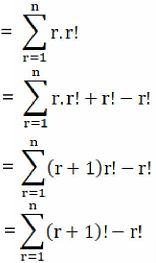= (2! – 1!) + (3! – 2!) + (4! – 3!) + ……… + (n! – (n – 1)!) + ((n+1)! – n!)

= 2! – 1! + 3! – 2! + 4! – 3! + ……… + n! – (n – 1)! + (n+1)! – n!

= (n + 1)! – 1!

= (n + 1)! – 1 [Since, P (n, n) = n!]

= P(n+1, n+1) – 1

= RHS

Hence Proved.

13. If P(15, r – 1) : P(16, r – 2) = 3 : 4, find r.

Solution:

Given:

P(15, r – 1) : P(16, r – 2) = 3 : 4

P(15, r – 1) / P(16, r – 2) = 3/4

By using the formula,

P (n, r) = n!/(n – r)!

P (15, r – 1) = 15! / (15 – r + 1)!

= 15! / (16 – r)!

P (16, r – 2) = 16!/(16 – r + 2)!

= 16!/(18 – r)!

So, from the question,

P(15, r – 1) / P(16, r – 2) = 3/4

Substituting the obtained values in above expression we get,

[15! / (16 – r)!] / [16!/(18 – r)!] = 3/4

[15! / (16 – r)!] × [(18 – r)! / 16!] = 3/4

[15! / (16 – r)!] × [(18 – r) (18 – r – 1) (18 – r – 2)!]/(16×15!) = 3/4

1/(16 – r)! × [(18 – r) (17 – r) (16 – r)!]/16 = 3/4

(18 – r) (17 – r) = 3/4 × 16

(18 – r) (17 – r) = 12

306 – 18r – 17r + r2 = 12

306 – 12 – 35r + r2 = 0

r2 – 35r + 294 = 0

r2 – 21r – 14r + 294 = 0

r(r – 21) – 14(r – 21) = 0

(r – 14) (r – 21) = 0

r = 14 or 21

For, P(n, r): r ≤ n

∴ r = 14 [for, P(15, r – 1)]

14. n+5Pn+1 = 11(n – 1)/2 n+3Pn, find n.

Solution:

Given:

n+5Pn+1 = 11(n – 1)/2 n+3Pn

P (n +5, n + 1) = 11(n – 1)/2 P(n + 3, n)

By using the formula,

P (n, r) = n!/(n – r)!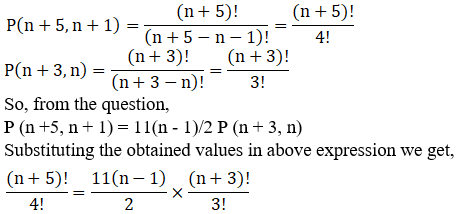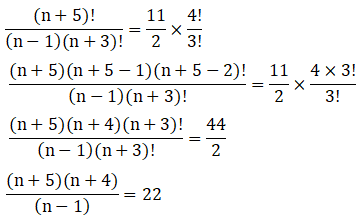(n + 5) (n + 4) = 22 (n – 1)

n2 + 4n + 5n + 20 = 22n – 22

n2 + 9n + 20 – 22n + 22 = 0

n2 – 13n + 42 = 0

n2 – 6n – 7n + 42 = 0

n(n – 6) – 7(n – 6) = 0

(n – 7) (n – 6) = 0

n = 7 or 6

∴ The value of n can either be 6 or 7.

15. In how many ways can five children stand in a queue?

Solution:

Number of arrangements of ‘n’ things taken all at a time = P (n, n)

So by using the formula,

By using the formula,

P (n, r) = n!/(n – r)!

The total number of ways in which five children can stand in a queue = the number of arrangements of 5 things taken all at a time = P (5, 5)

So,

P (5, 5) = 5!/(5 – 5)!

= 5!/0!

= 5! [Since, 0! = 1]

= 5 × 4 × 3 × 2 × 1

= 120

Hence, Number of ways in which five children can stand in a queue are 120.

16. From among the 36 teachers in a school, one principal and one vice-principal are to be appointed. In how many ways can this be done?

Solution:

Given:

The total number of teachers in a school = 36

We know, number of arrangements of n things taken r at a time = P(n, r)

By using the formula,

P (n, r) = n!/(n – r)!

∴ The total number of ways in which this can be done = the number of arrangements of 36 things taken 2 at a time = P(36, 2)

P (36, 2) = 36!/(36 – 2)!

= 36!/34!

= (36 × 35 × 34!)/34!

= 36 × 35

= 1260

Hence, Number of ways in which one principal and one vice-principal are to be appointed out of total 36 teachers in school are 1260.

EXERCISE 16.4 PAGE NO: 16.36

1. In how many ways can the letters of the word ‘FAILURE’ be arranged so that the consonants may occupy only odd positions?

Solution:

Given:

The word ‘FAILURE’

Number of vowels in word ‘FAILURE’ = 4(E, A, I, U)

Number of consonants = 3(F, L, R)

Let consonants be denoted by C

Odd positions are 1, 3, 5 or 7

The consonants can be arranged in these 4 odd places in 4P3 ways.

Remaining 3 even places (2, 4, 6) are to be occupied by the 4 vowels. This can be done in 4P3 ways.

So, the total number of words in which consonants occupy odd places = 4P3 × 4P3

By using the formula,

P (n, r) = n!/(n – r)!

P (4, 3) × P (4, 3) = 4!/(4 – 3)! × 4!/(4 – 3)!

= 4 × 3 × 2 × 1 × 4 × 3 × 2 × 1

= 24 × 24

= 576

Hence, the number of arrangements so that the consonants occupy only odd positions is 576.

2. In how many ways can the letters of the word ‘STRANGE’ be arranged so that
(i) the vowels come together?

(ii) the vowels never come together? And

(iii) the vowels occupy only the odd places?

Solution:

Given:

The word ‘STRANGE’

There are 7 letters in the word ‘STRANGE’, which includes 2 vowels (A,E) and 5 consonants (S,T,R,N,G).

(i) the vowels come together?

Considering 2 vowels as one letter so we will have 6 letters which can be arranged in 6P6 ways.

(A,E) can be put together in 2P2 ways.

Hence, the required number of words are

By using the formula,

P (n, r) = n!/(n – r)!

P (6, 6) × P (2, 2) = 6!/(6 – 6)! × 2!/(2 – 2)!

= 6! × 2!

= 6 × 5 × 4 × 3 × 2 × 1 × 2 × 1

= 720 × 2

= 1440

Hence, total number of arrangements in which vowels come together is 1440.

(ii) the vowels never come together?

The total number of letters in the word ‘STRANGE’ is 7P7 = 7! = 7 × 6 × 5 × 4 × 3 × 2 × 1 = 5040

So,

Total number of words in which vowels never come together = total number of words – number of words in which vowels are always together

= 5040 – 1440

= 3600

Hence, the total number of arrangements in which vowel never come together is 3600.

(iii) the vowels occupy only the odd places?

There are 7 letters in the word ‘STRANGE’. Out of these letters (A,E) are the vowels.

There are 4 odd places in the word ‘STRANGE’. The two vowels can be arranged in 4P2 ways.

The remaining 5 consonants an be arranged among themselves in 5P5 ways.

So, the total number of arrangements is

By using the formula,

P (n, r) = n!/(n – r)!

P (4, 2) × P (5, 5) = 4!/(4 – 2)! × 5!/(5 – 5)!

= 4!/2! × 5!

= (4×3×2!)/2! × 5!

= 4×3 × 5 × 4 × 3 × 2 × 1

= 12 × 120

= 1440

Hence, the number of arrangements so that the vowels occupy only odd positions is 1440.

3. How many words can be formed from the letters of the word ‘SUNDAY’? How many of these begin with D?

Solution:

Given:

The word ‘SUNDAY’

Total number of letters in the word ‘SUNDAY’ is 6.

So, number of arrangements of 6 things, taken all at a time is 6P6

= 6! = 6 × 5 × 4 × 3 × 2 × 1 = 720

Now, we shall find the number of words starting with D

So let’s fix the first position with letter D, then remaining number of letters is 5.

The number of arrangements of 5 things, taken all at a time is 5P5 = 5! = 5 × 4 × 3 × 2 × 1 = 120

Hence, the total number of words can be made by letters of the word ‘SUNDAY’ is 720.

The possible number of words using letters of ‘SUNDAY’ starting with ‘D’ is 120.

4. How many words can be formed out of the letters of the word, ‘ORIENTAL,’ so that the vowels always occupy the odd places?

Solution:

Given:

The word ‘ORIENTAL’

Number of vowels in the word ‘ORIENTAL’ = 4(O, I, E, A)

Number of consonants in given word = 4(R, N, T, L)

Odd positions are (1, 3, 5 or 7)

Four vowels can be arranged in these 4 odd places in 4P4 ways.

Remaining 4 even places (2,4,6,8) are to be occupied by the 4 consonants in 4P4 ways.

So, by using the formula,

P (n, r) = n!/(n – r)!

P (4, 4) × P (4, 4) = 4!/(4 – 4)! × 4!/(4 – 4)!

= 4! × 4!

= 4 × 3 × 2 × 1 × 4 × 3 × 2 × 1

= 24 × 24

= 576

Hence, the number of arrangements so that the vowels occupy only odd positions is 576.

5. How many different words can be formed with the letters of word ‘SUNDAY’? How many of the words begin with N? How many begin with N and end in Y?

Solution:

Given:

The word ‘SUNDAY’

Total number of letters in the word ‘SUNDAY’ is 6.

So, number of arrangements of 6 things, taken all at a time is 6P6

= 6! = 6 × 5 × 4 × 3 × 2 × 1 = 720

Now, we shall find the number of words starting with N

So let’s fix the first position with letter N, then remaining number of letters is 5.

The number of arrangements of 5 things, taken all at a time is 5P5 = 5! = 5 × 4 × 3 × 2 × 1 = 120

Now, we need to find out a number of words starting with N and ending with Y

So let’s fix the first position with letter N and Y at the end, then remaining number of letters is 4 which can be arranged in 4P4 ways. = 4! = 4 × 3 × 2 × 1 = 24

Hence, the total number of words can be made by letters of the word ‘SUNDAY’ is 720.

The possible number of words using letters of ‘SUNDAY’ starting with ‘N’ is 120.

The possible number of words using letters of ‘SUNDAY’ starting with ‘N’ and ending with ‘Y’ is 24.

6. How many different words can be formed from the letters of the word ‘GANESHPURI’? In how many of these words:
(i) the letter G always occupies the first place?

(ii) the letter P and I respectively occupy the first and last place?

(iii) Are the vowels always together?

(iv) the vowels always occupy even places?

Solution:

Given:

The word ‘GANESHPURI’

There are 10 letters in the word ‘GANESHPURI’. The total number of words formed is 10P10 = 10!

(i) the letter G always occupies the first place?

If we fix the first position with letter G, then remaining number of letters is 9.

The number of arrangements of 9 things, taken all at a time is 9P9 = 9! Ways.

Hence, a possible number of words using letters of ‘GANESHPURI’ starting with ‘G’ is 9!

(ii) the letter P and I respectively occupy the first and last place?

If we fix the first position with letter P and I in the end, then remaining number of letters is 8.

The number of arrangements of 8 things, taken all at a time is 8P8 = 8! Ways.

Hence, a possible number of words using letters of ‘GANESHPURI’ starting with ‘P’ and ending with ‘I’ is 8!

(iii) Are the vowels always together?

There are 4 vowels and 6 consonants in the word ‘GANESHPURI’.

Consider 4 (A,E,I,U) vowels as one letter, then total number of letters is 7 (A,E,I,U, G, N, S, H , P, R)

The number of arrangements of 7 things, taken all at a time is 7P7 = 7! Ways.

(A, E, I, U) can be put together in 4! Ways.

Hence, total number of arrangements in which vowels come together is 7! × 4!

(iv) the vowels always occupy even places?

Number of vowels in the word ‘GANESHPURI’ = 4(A, E, I, U)

Number of consonants = 6(G, N, S, H, R, I)

Even positions are 2, 4, 6, 8 or 10

Now, we have to arrange 10 letters in a row such that vowels occupy even places. There are 5 even places (2, 4, 6, 8 or 10). 4 vowels can be arranged in these 5 even places in 5P4 ways.

Remaining 5 odd places (1, 3, 5, 7, 9) are to be occupied by the 6 consonants in 6P5 ways.

So, by using the formula,

P (n, r) = n!/(n – r)!

P (5, 4) × P (6, 5) = 5!/(5 – 4)! × 6!/(6 – 5)!

= 5! × 6!

Hence, number of arrangements so that the vowels occupy only even positions is 5! × 6!

EXERCISE 16.5 PAGE NO: 16.42

1. Find the number of words formed by permuting all the letters of the following words :
(i) INDEPENDENCE

(ii) INTERMEDIATE

(iii) ARRANGE

(iv) INDIA

(v) PAKISTAN

(vi) RUSSIA

(vii) SERIES

(viii) EXERCISES

(ix) CONSTANTINOPLE

Solution:

(i) INDEPENDENCE

There are 12 letters in the word ‘INDEPENDENCE’ out of which 2 are D’s, 3 are N’s, 4 are E’s and the rest all are distinct.

So by using the formula,

n!/ (p! × q! × r!)

total number of arrangements = 12! / (2! 3! 4!)

= [12×11×10×9×8×7×6×5×4×3×2×1] / (2! 3! 4!)

= [12×11×10×9×8×7×6×5] / (2×1×3×2×1)

= 11×10×9×8×7×6×5

= 1663200

(ii) INTERMEDIATE

There are 12 letters in the word ‘INTERMEDIATE’ out of which 2 are I’s, 2 are T’s, 3 are E’s and the rest all are distinct.

So by using the formula,

n!/ (p! × q! × r!)

total number of arrangements = 12! / (2! 2! 3!)

= [12×11×10×9×8×7×6×5×4×3×2×1] / (2! 2! 3!)

= [12×11×10×9×8×7×6×5×3×2×1] / (3!)

= 12×11×10×9×8×7×6×5

= 19958400

(iii) ARRANGE

There are 7 letters in the word ‘ARRANGE’ out of which 2 are A’s, 2 are R’s and the rest all are distinct.

So by using the formula,

n!/ (p! × q! × r!)

total number of arrangements = 7! / (2! 2!)

= [7×6×5×4×3×2×1] / (2! 2!)

= 7×6×5×3×2×1

= 1260

(iv) INDIA

There are 5 letters in the word ‘INDIA’ out of which 2 are I’s and the rest all are distinct.

So by using the formula,

n!/ (p! × q! × r!)

total number of arrangements = 5! / (2!)

= [5×4×3×2×1] / 2!

= 5×4×3

= 60

(v) PAKISTAN

There are 8 letters in the word ‘PAKISTAN’ out of which 2 are A’s and the rest all are distinct.

So by using the formula,

n!/ (p! × q! × r!)

total number of arrangements = 8! / (2!)

= [8×7×6×5×4×3×2×1] / 2!

= 8×7×6×5×4×3

= 20160

(vi) RUSSIA

There are 6 letters in the word ‘RUSSIA’ out of which 2 are S’s and the rest all are distinct.

So by using the formula,

n!/ (p! × q! × r!)

total number of arrangements = 6! / (2!)

= [6×5×4×3×2×1] / 2!

= 6×5×4×3

= 360

(vii) SERIES

There are 6 letters in the word ‘SERIES’ out of which 2 are S’s, 2 are E’s and the rest all are distinct.

So by using the formula,

n!/ (p! × q! × r!)

total number of arrangements = 6! / (2! 2!)

= [6×5×4×3×2×1] / (2! 2!)

= 6×5×3×2×1

= 180

(viii) EXERCISES

There are 9 letters in the word ‘EXERCISES’ out of which 3 are E’s, 2 are S’s and the rest all are distinct.

So by using the formula,

n!/ (p! × q! × r!)

total number of arrangements = 9! / (3! 2!)

= [9×8×7×6×5×4×3×2×1] / (3! 2!)

= [9×8×7×6×5×4×3×2×1] / (3×2×1×2×1)

= 9×8×7×5×4×3×1

= 30240

(ix) CONSTANTINOPLE

There are 14 letters in the word ‘CONSTANTINOPLE’ out of which 2 are O’s, 3 are N’s, 2 are T’s and the rest all are distinct.

So by using the formula,

n!/ (p! × q! × r!)

total number of arrangements = 14! / (2! 3! 2!)

= 14!/ (2×1×3×2×1×2×1)

= 14! / 24

2. In how many ways can the letters of the word ‘ALGEBRA’ be arranged without changing the relative order of the vowels and consonants?

Solution:

Given:

The word ‘ALGEBRA’

There are 4 consonants in the word ‘ALGEBRA’

The number of ways to arrange these consonants is 4P4 = 4!

There are 3 vowels in the word ‘ALGEBRA’ of which, 2 are A’s

So vowels can be arranged in n!/ (p! × q! × r!) = 3! / 2! Ways

Hence, the required number of arrangements = 4! × (3! / 2!)

= [4×3×2×1×3×2×1] / (2×1)

= 4×3×2×1×3 = 72

3. How many words can be formed with the letters of the word ‘UNIVERSITY,’ the vowels remaining together?

Solution:

Given:

The word ‘UNIVERSITY’

There are 10 letters in the word ‘UNIVERSITY’ out of which 2 are I’s

There are 4 vowels in the word ‘UNIVERSITY’ out of which 2 are I’s

So these vowels can be put together in n!/ (p! × q! × r!) = 4! / 2! Ways

Let us consider these 4 vowels as one letter, remaining 7 letters can be arranged in 7! Ways.

Hence, the required number of arrangements = (4! / 2!) × 7!

= (4×3×2×1×7×6×5×4×3×2×1) / (2×1)

= 4×3×2×1×7×6×5×4×3

= 60480

4. Find the total number of arrangements of the letters in the expression a3 b2 c4 when written at full length.

Solution:

There are 9 (i.e powers 3 + 2 + 4 = 9) objects in the expression a3 b2 c4 and there are 3 a’s, 2 b’s, 4 c’s

So by using the formula,

n!/ (p! × q! × r!)

total number of arrangements = 9! / (3! 2! 4!)

= [9×8×7×6×5×4×3×2×1] / (3×2×1×2×1×4×3×2×1)

= 7×6×5×3×2×1

= 1260

5. How many words can be formed with the letters of the word ‘PARALLEL’ so that all L’s do not come together?

Solution:

Given:

The word ‘PARALLEL’

There are 8 letters in the word ‘PARALLEL’ out of which 2 are A’s, 3 are L’s and the rest all are distinct.

So by using the formula,

n!/ (p! × q! × r!)

total number of arrangements = 8! / (2! 3!)

= [8×7×6×5×4×3×2×1] / (2×1×3×2×1)

= 8×7×5×4×3×1

= 3360

Now, let us consider all L’s together as one letter, so we have 6 letters out of which A repeats 2 times and others are distinct.

These 6 letters can be arranged in 6! / 2! Ways.

The number of words in which all L’s come together = 6! / 2!

= [6×5×4×3×2×1] / (2×1)

= 6×5×4×3

= 360

So, now the number of words in which all L’s do not come together = total number of arrangements – The number of words in which all L’s come together

= 3360 – 360 = 3000

6. How many words can be formed by arranging the letters of the word ‘MUMBAI’ so that all M’s come together?

Solution:

Given:

The word ‘MUMBAI’

There are 6 letters in the word ‘MUMBAI’ out of which 2 are M’s and the rest all are distinct.

So let us consider both M’s together as one letter, the remaining 5 letters can be arranged in 5! Ways.

Total number of arrangements = 5!

= 5×4×3×2×1

= 120

Hence, a total number of words formed during the arrangement of letters of word MUMBAI such that all M’s remains together equals to 120.

7. How many numbers can be formed with the digits 1, 2, 3, 4, 3, 2, 1 so that the odd digits always occupy the odd places?

Solution:

Given:

The digits 1, 2, 3, 4, 3, 2, 1

The total number of digits are 7.

There are 4 odd digits 1,1,3,3 and 4 odd places (1,3,5,7)

So, the odd digits can be arranged in odd places in n!/ (p! × q! × r!) = 4!/(2! 2!) ways.

The remaining even digits 2,2,4 can be arranged in 3 even places in n!/ (p! × q! × r!) = 3!/2! Ways.

Hence, the total number of digits = 4!/(2! 2!) × 3!/2!

= [4×3×2×1×3×2×1] / (2! 2! 2!)

= 3×2×1×3×1

= 18

Hence, the number of ways of arranging the digits such odd digits always occupies odd places is equals to 18.

8. How many different signals can be made from 4 red, 2 white, and 3 green flags by arranging all of them vertically on a flagstaff?

Solution:

Given:

Number of red flags = 4

Number of white flags = 2

Number of green flags = 3

So there are total 9 flags, out of which 4 are red, 2 are white, 3 are green

By using the formula,

n!/ (p! × q! × r!) = 9! / (4! 2! 3!)

= [9×8×7×6×5×4!] / (4!×2×1×3×2×1)

= [9×8×7×6×5] / (2×3×2)

= 9×4×7×5

= 1260

Hence, 1260 different signals can be made.

9. How many numbers of four digits can be formed with the digits 1, 3, 3, 0?

Solution:

Given:

The digits 1, 3, 3, 0

Total number of digits = 4

Digits of the same type = 2

Total number of 4 digit numbers = 4! / 2!

Where, zero cannot be the first digit of the four digit numbers.

So, Total number of 3 digit numbers = 3! / 2!

Total number of Numbers = (4! / 2!) – (3! / 2!)

= [(4×3×2)/2] – [(3×2)/2]

= [4×3] – 

= 12 – 3

= 9

Hence, total number of four digit can be formed is 9.

10. In how many ways can the letters of the word ‘ARRANGE’ be arranged so that the two R’s are never together?

Solution:

There are 7 letters in the word ‘ARRANGE’ out of which 2 are A’s, 2 are R’s and the rest all are distinct.

So by using the formula,

n!/ (p! × q! × r!)

total number of arrangements = 7! / (2! 2!)

= [7×6×5×4×3×2×1] / (2! 2!)

= 7×6×5×3×2×1

= 1260

Let us consider all R’s together as one letter, there are 6 letters remaining. Out of which 2 times A repeats and others are distinct.

So these 6 letters can be arranged in n!/ (p! × q! × r!) = 6!/2! Ways.

The number of words in which all R’s come together = 6! / 2!

= [6×5×4×3×2!] / 2!

= 6×5×4×3

= 360

So, now the number of words in which all L’s do not come together = total number of arrangements – The number of words in which all L’s come together

= 1260 – 360

= 900

Hence, the total number of arrangements of word ARRANGE in such a way that not all R’s come together is 900.

11. How many different numbers, greater than 50000 can be formed with the digits 0, 1, 1, 5, 9.

Solution:

Given:

The digits 0, 1, 1, 5, 9

Total number of digits = 5

So now, number greater than 50000 will have either 5 or 9 in the first place and will consists of 5 digits.

Number of 5 digit numbers at first place = 4! / 2! [Since, 1 is repeated]

= [4×3×2!] / 2!

= 4 × 3

= 12

Similarly, number of 9 digit numbers at first place = 4! / 2! = 12

The required number of Numbers = 12 + 12 = 24

Hence, 12 different numbers can be formed.

12. How many words can be formed from the letters of the word ‘SERIES’ which start with S and end with S?

Solution:

Given:

The word ‘SERIES’

There are 6 letters in the word ‘SERIES’ out of which 2 are S’s, 2 are E’s and the rest all are distinct.

Now, Let us fix 5 letters at the extreme left and also at the right end. So we are left with 4 letters of which 2 are E’s.

These 4 letters can be arranged in n!/ (p! × q! × r!) = 4! / 2! Ways.

Required number of arrangements is = 4! / 2!

= [4×3×2!] / 2!

= 4 × 3

= 12

Hence, a total number of arrangements of the letters of the word ‘SERIES’ in such a way that the first and last position is always occupied by the letter S is 12.

13. How many permutations of the letters of the word ‘MADHUBANI’ do not begin with M but end with I?

Solution:

Given:

Total number of letters = 9

A total number of arrangements of word MADHUBANI excluding I: Total letters 8. Repeating letter A, repeating twice.

The total number of arrangements that end with letter I = 8! / 2!

= [8×7×6×5×4×3×2!] / 2!

= 8×7×6×5×4×3

= 20160

If the word start with ‘M’ and end with ‘I’, there are 7 places for 7 letters.

The total number of arrangements that start with ‘M’ and end with letter I = 7! / 2!

= [7×6×5×4×3×2!] / 2!

= 7×6×5×4×3

= 2520

The total number of arrangements that do not start with ‘M’ but end with letter I = The total number of arrangements that end with letter I – The total number of arrangements that start with ‘M’ and end with letter I

= 20160 – 2520

= 17640

Hence, a total number of arrangements of word MADHUBANI in such a way that the word is not starting with M but ends with I is 17640.

14. Find the number of numbers, greater than a million that can be formed with the digit 2, 3, 0, 3, 4, 2, 3.

Solution:

Given:

The digits 2, 3, 0, 3, 4, 2, 3

Total number of digits = 7

We know, zero cannot be the first digit of the 7 digit numbers.

Number of 6 digit number = n!/ (p! × q! × r!) = 6! / (2! 3!) Ways. [2 is repeated twice and 3 is repeated 3 times]

The total number of arrangements = 6! / (2! 3!)

= [6×5×4×3×2×1] / (2×3×2)

= 5×4×3×1

= 60

Now, number of 7 digit number = n!/ (p! × q! × r!) = 7! / (2! 3!) Ways

The total number of arrangements = 7! / (2! 3!)

= [7×6×5×4×3×2×1] / (2×3×2)

= 7×5×4×3×1

= 420

So, total numbers which is greater than 1 million = 420 – 60 = 360

Hence, total number of arrangements of 7 digits (2, 3, 0, 3, 4, 2, 3) forming a 7 digit number is 360.

### Also, access exercises of RD Sharma Solutions for Class 11 Maths Chapter 16 – Permutations

Exercise 16.1 Solutions

Exercise 16.2 Solutions

Exercise 16.3 Solutions

Exercise 16.4 Solutions

Exercise 16.5 Solutions

## Frequently Asked Questions on RD Sharma Solutions for Class 11 Maths Chapter 16

### Is the RD Sharma Solutions for Class 11 Maths Chapter 16 the best reference guide for the students?

To prepare well for the board exams, students should choose the perfect reference guide which helps them to grasp the concepts effortlessly. The RD Sharma Solutions can be referred to while answering the textbook questions to get a clear idea about the concepts which are important from the exam perspective. For this purpose, the teachers at BYJU’S have prepared the chapter wise solutions to guide the students.

### Why should I refer to the BYJU’S RD Sharma Solutions for Class 11 Maths Chapter 16?

The subject experts at BYJU’S have formulated the RD Sharma Solutions for Class 11 Maths Chapter 16 to help students understand the concepts efficiently. The solutions are completely based on the latest syllabus and guidelines of the CBSE board. Simple steps are used in creating the solutions which makes it easy for the students while learning. Shortcut tips and techniques are provided to remember important topics and are highlighted with the aim of helping the students to score more marks in the board exams.

### Where can I get the RD Sharma Solutions for Class 11 Maths Chapter 16 online?

Class 11 is an important stage in every student’s life. The faculty at BYJU’S have created the solutions based on the latest CBSE syllabus. Students can download the solutions available in PDF format from BYJU’S website and score high marks in the board exams. The solutions are designed keeping in mind the exam preparation of students irrespective of their intelligence quotient.